# Confirmatory Factor Analysis, Latent Variable Models, and Structural Equation Modeling

## Packages:

• lavaan
• semPlot These install a lot of additional packages for analysis and visualization.

Exploratory factor analysis (EFA) can be used to discover common underlying factors. But sometimes you might have hypotheses about the factor structure, and want to either test or confirm this in your data. The particular factor structure that come out of EFA might be the best bottom-up description, but one associated with a particular theory may be nearly as good, or perhaps even equally good, and it might be better than a description related to an alternate theory. For example, in PCA, we saw a data set created so that factors of visual and verbal intelligence emerged. The solution showed two factors, but one related to general intelligence and one that was a difference between visual and verbal. When we applied exploratory factor analysis, a rotation changed this to map more closely onto our theory, but what if we’d like to identify a particular structure (or set of alternative structures) and determine whether the data are well described by this structure? We can do this with a set of methods known as confirmatory factor analysis, structure equation modeling, and other advanced related methods.

Methods for doing this in the context of factor analysis are often called confirmatory factor analysis. However, these probably only properly apply to independent factor models. That is, you could create multiple specific models that look like regression equations, but the predictor variable is a latent, hidden variable (much like mixture-modeling). But researchers found that their theoretical models were more complex than this, and the same machinery that permits inferring latent factors can infer much more sit models that determine a more complex structure. There are many varieties of these that go under many names; most commonly structure equation models (SEM), latent variable models, path analysis models, and specific applications like latent growth models. Furthermore, advances in hierarchical Bayes models provides substantial ability to infer latent structures. Many of these models (especially the SEM variety) still rely on correlation matrices for the data, and so even those that claim to unearth na causal structure are basing this on correlations, and are really not able to make causal inferences (this relies on experimental design, not statistical inference).

# Confirmatory Factor Analysis Example

Confirmatory factor analysis (CFA) is a way to create a latent structure and test it (usually against another latent structure) in order to compare hypotheses. Generally, you will specify the factors loading onto variables. These variables are independent factors. You can compare different numbers of factors, or different loadings. This is useful if you do a follow-up experiment after a first experiment where you conducted an EFA to identify general factors.

We will use the lavaan library for this, although other free and commercial projects exist. We will start by looking at the built-in confirmatory factor analysis example in lavaan:

library(lavaan)  ##needed for holzingerswineford data
data <- dplyr::select(HolzingerSwineford1939, x1:x9)
cc <- cor(data)
pca <- princomp(cc)
loadings(pca)

Comp.1 Comp.2 Comp.3 Comp.4 Comp.5 Comp.6 Comp.7 Comp.8 Comp.9
x1         0.290  0.607         0.566  0.419                0.169
x2         0.522 -0.506  0.379                      -0.183  0.529
x3 -0.136  0.436  0.336 -0.549 -0.276 -0.410                0.356
x4  0.498 -0.145  0.101                0.106 -0.428 -0.715
x5  0.519 -0.184         0.175        -0.162 -0.313  0.609  0.399
x6  0.486                             -0.238  0.815 -0.142
x7 -0.273 -0.532        -0.318         0.413  0.169         0.576
x8 -0.322 -0.320  0.113  0.302  0.461 -0.610        -0.203  0.241
[ reached getOption("max.print") -- omitted 1 row ]

Comp.1 Comp.2 Comp.3 Comp.4 Comp.5 Comp.6 Comp.7 Comp.8 Comp.9
Proportion Var  0.111  0.111  0.111  0.111  0.111  0.111  0.111  0.111  0.111
Cumulative Var  0.111  0.222  0.333  0.444  0.556  0.667  0.778  0.889  1.000
f <- factanal(data, factors = 3)

# f2 <- fa:fa(data,factors=3)
library(lavaan)

library(semPlot)  #this won't compile for me
example(cfa)

cfa> ## The famous Holzinger and Swineford (1939) example
cfa> HS.model <- ' visual  =~ x1 + x2 + x3
cfa+               textual =~ x4 + x5 + x6
cfa+               speed   =~ x7 + x8 + x9 '

cfa> fit <- cfa(HS.model, data = HolzingerSwineford1939)

cfa> summary(fit, fit.measures = TRUE)
lavaan 0.6-8 ended normally after 35 iterations

Estimator                                         ML
Optimization method                           NLMINB
Number of model parameters                        21

Number of observations                           301

Model Test User Model:

Test statistic                                85.306
Degrees of freedom                                24
P-value (Chi-square)                           0.000

Model Test Baseline Model:

Test statistic                               918.852
Degrees of freedom                                36
P-value                                        0.000

User Model versus Baseline Model:

Comparative Fit Index (CFI)                    0.931
Tucker-Lewis Index (TLI)                       0.896

Loglikelihood and Information Criteria:

Loglikelihood user model (H0)              -3737.745
Loglikelihood unrestricted model (H1)      -3695.092

Akaike (AIC)                                7517.490
Bayesian (BIC)                              7595.339

Root Mean Square Error of Approximation:

RMSEA                                          0.092
90 Percent confidence interval - lower         0.071
90 Percent confidence interval - upper         0.114
P-value RMSEA <= 0.05                          0.001

Standardized Root Mean Square Residual:

SRMR                                           0.065

Parameter Estimates:

Standard errors                             Standard
Information                                 Expected
Information saturated (h1) model          Structured

Latent Variables:
Estimate  Std.Err  z-value  P(>|z|)
visual =~
x1                1.000
x2                0.554    0.100    5.554    0.000
x3                0.729    0.109    6.685    0.000
textual =~
x4                1.000
x5                1.113    0.065   17.014    0.000
x6                0.926    0.055   16.703    0.000
speed =~
x7                1.000
x8                1.180    0.165    7.152    0.000
x9                1.082    0.151    7.155    0.000

Covariances:
Estimate  Std.Err  z-value  P(>|z|)
visual ~~
textual           0.408    0.074    5.552    0.000
speed             0.262    0.056    4.660    0.000
textual ~~
speed             0.173    0.049    3.518    0.000

Variances:
Estimate  Std.Err  z-value  P(>|z|)
.x1                0.549    0.114    4.833    0.000
.x2                1.134    0.102   11.146    0.000
.x3                0.844    0.091    9.317    0.000
.x4                0.371    0.048    7.779    0.000
.x5                0.446    0.058    7.642    0.000
.x6                0.356    0.043    8.277    0.000
.x7                0.799    0.081    9.823    0.000
.x8                0.488    0.074    6.573    0.000
.x9                0.566    0.071    8.003    0.000
visual            0.809    0.145    5.564    0.000
textual           0.979    0.112    8.737    0.000
speed             0.384    0.086    4.451    0.000

let’s break down this. This uses a data set HolzingerSwineford1939. This data set has nine measures that measure a number of abilties:

• x1: visual perception
• x2: cubes test
• x3: lozenges test
• x4: paragraph comprehension
• x5: sentence completion
• x6: word meaning
• x8: speeded counting of dots
• x9: speeded discrimination of letters

These have often been thought of having three main components: visual (x1.3), text (x4.6) and speed (x7:9).

help(HolzingerSwineford1939)

The name lavaan refers to latent variable analysis, which is the essence of confirmatory factor analysis. This is similar to the latent variables we used in mixture modeling (hidden group membership), as well as latent variables used in item response theory.

For simple confirmatory factor analysis, you can think of it as a set of regressions, but instead of an outcome variable, we relate observable measures to a latent variable. For lavaan, we specify a model using a special text markup that isn’t exactly R code. Enter the latent variable names on the left, the observed names on the right, separated with =~, and with each factor separated by a line break. Then, you can use the cfa function to fit it using a specified data set. The =~ operator indicates a relationship “is manifested by”, which means that the variable on the right need to actually measured. There are other relationships that you might use in more complex models.

HS.model <- " visual   =~ x2 + x1 + x3
textual  =~ x4 + x5 + x6
speed    =~ x7 + x8 + x9 "

fit <- cfa(HS.model, data = HolzingerSwineford1939)
fit
lavaan 0.6-8 ended normally after 38 iterations

Estimator                                         ML
Optimization method                           NLMINB
Number of model parameters                        21

Number of observations                           301

Model Test User Model:

Test statistic                                85.306
Degrees of freedom                                24
P-value (Chi-square)                           0.000
summary(fit, fit.measures = T)
lavaan 0.6-8 ended normally after 38 iterations

Estimator                                         ML
Optimization method                           NLMINB
Number of model parameters                        21

Number of observations                           301

Model Test User Model:

Test statistic                                85.306
Degrees of freedom                                24
P-value (Chi-square)                           0.000

Model Test Baseline Model:

Test statistic                               918.852
Degrees of freedom                                36
P-value                                        0.000

User Model versus Baseline Model:

Comparative Fit Index (CFI)                    0.931
Tucker-Lewis Index (TLI)                       0.896

Loglikelihood and Information Criteria:

Loglikelihood user model (H0)              -3737.745
Loglikelihood unrestricted model (H1)      -3695.092

Akaike (AIC)                                7517.490
Bayesian (BIC)                              7595.339

Root Mean Square Error of Approximation:

RMSEA                                          0.092
90 Percent confidence interval - lower         0.071
90 Percent confidence interval - upper         0.114
P-value RMSEA <= 0.05                          0.001

Standardized Root Mean Square Residual:

SRMR                                           0.065

Parameter Estimates:

Standard errors                             Standard
Information                                 Expected
Information saturated (h1) model          Structured

Latent Variables:
Estimate  Std.Err  z-value  P(>|z|)
visual =~
x2                1.000
x1                1.807    0.325    5.554    0.000
x3                1.318    0.239    5.509    0.000
textual =~
x4                1.000
x5                1.113    0.065   17.014    0.000
x6                0.926    0.055   16.703    0.000
speed =~
x7                1.000
x8                1.180    0.165    7.152    0.000
x9                1.082    0.151    7.155    0.000

Covariances:
Estimate  Std.Err  z-value  P(>|z|)
visual ~~
textual           0.226    0.052    4.329    0.000
speed             0.145    0.038    3.864    0.000
textual ~~
speed             0.173    0.049    3.518    0.000

Variances:
Estimate  Std.Err  z-value  P(>|z|)
.x2                1.134    0.102   11.146    0.000
.x1                0.549    0.114    4.833    0.000
.x3                0.844    0.091    9.317    0.000
.x4                0.371    0.048    7.778    0.000
.x5                0.446    0.058    7.642    0.000
.x6                0.356    0.043    8.277    0.000
.x7                0.799    0.081    9.823    0.000
.x8                0.488    0.074    6.573    0.000
.x9                0.566    0.071    8.003    0.000
visual            0.248    0.077    3.214    0.001
textual           0.979    0.112    8.737    0.000
speed             0.384    0.086    4.451    0.000

The above model should produce the same model that the ‘example’ did.

HS.model2 <- " visual   =~ x1 + x2 + x3 + x4 + x5 + x6
speed    =~ x7 + x8 + x9 "
fit2 <- cfa(HS.model2, data = HolzingerSwineford1939)
fit2
lavaan 0.6-8 ended normally after 35 iterations

Estimator                                         ML
Optimization method                           NLMINB
Number of model parameters                        19

Number of observations                           301

Model Test User Model:

Test statistic                               181.337
Degrees of freedom                                26
P-value (Chi-square)                           0.000
summary(fit2, fit.measures = T)
lavaan 0.6-8 ended normally after 35 iterations

Estimator                                         ML
Optimization method                           NLMINB
Number of model parameters                        19

Number of observations                           301

Model Test User Model:

Test statistic                               181.337
Degrees of freedom                                26
P-value (Chi-square)                           0.000

Model Test Baseline Model:

Test statistic                               918.852
Degrees of freedom                                36
P-value                                        0.000

User Model versus Baseline Model:

Comparative Fit Index (CFI)                    0.824
Tucker-Lewis Index (TLI)                       0.756

Loglikelihood and Information Criteria:

Loglikelihood user model (H0)              -3785.760
Loglikelihood unrestricted model (H1)      -3695.092

Akaike (AIC)                                7609.521
Bayesian (BIC)                              7679.956

Root Mean Square Error of Approximation:

RMSEA                                          0.141
90 Percent confidence interval - lower         0.122
90 Percent confidence interval - upper         0.161
P-value RMSEA <= 0.05                          0.000

Standardized Root Mean Square Residual:

SRMR                                           0.113

Parameter Estimates:

Standard errors                             Standard
Information                                 Expected
Information saturated (h1) model          Structured

Latent Variables:
Estimate  Std.Err  z-value  P(>|z|)
visual =~
x1                1.000
x2                0.509    0.156    3.254    0.001
x3                0.477    0.150    3.189    0.001
x4                1.992    0.272    7.314    0.000
x5                2.194    0.300    7.303    0.000
x6                1.852    0.254    7.294    0.000
speed =~
x7                1.000
x8                1.148    0.163    7.054    0.000
x9                0.891    0.124    7.189    0.000

Covariances:
Estimate  Std.Err  z-value  P(>|z|)
visual ~~
speed             0.095    0.029    3.287    0.001

Variances:
Estimate  Std.Err  z-value  P(>|z|)
.x1                1.112    0.093   11.922    0.000
.x2                1.318    0.108   12.193    0.000
.x3                1.219    0.100   12.196    0.000
.x4                0.373    0.047    7.853    0.000
.x5                0.474    0.059    8.059    0.000
.x6                0.351    0.043    8.221    0.000
.x7                0.732    0.083    8.821    0.000
.x8                0.428    0.082    5.201    0.000
.x9                0.658    0.071    9.300    0.000
visual            0.246    0.067    3.661    0.000
speed             0.451    0.095    4.733    0.000
anova(fit, fit2)
Chi-Squared Difference Test

Df    AIC    BIC   Chisq Chisq diff Df diff Pr(>Chisq)
fit  24 7517.5 7595.3  85.305
fit2 26 7609.5 7680.0 181.337     96.031       2  < 2.2e-16 ***
---
Signif. codes:  0 '***' 0.001 '**' 0.01 '*' 0.05 '.' 0.1 ' ' 1
partable(fit)
  id     lhs op rhs user block group free ustart exo label plabel start   est
1  1  visual =~  x2    1     1     1    0      1   0         .p1. 1.000 1.000
2  2  visual =~  x1    1     1     1    1     NA   0         .p2. 1.286 1.807
3  3  visual =~  x3    1     1     1    2     NA   0         .p3. 1.424 1.318
4  4 textual =~  x4    1     1     1    0      1   0         .p4. 1.000 1.000
5  5 textual =~  x5    1     1     1    3     NA   0         .p5. 1.133 1.113
se
1 0.000
2 0.325
3 0.239
4 0.000
5 0.065
[ reached 'max' / getOption("max.print") -- omitted 19 rows ]
# install.packages('semPlot',dependencies=T)
library(psych)
## library(semPlot) ##this won't install for me! semPaths(fit,
## 'model','est',curvePivot = FALSE,edge.label.cex = 0.5)

lavaan.diagram(fit, cut = 0.2, digits = 2)  ##in psych package
summary(fit, fit.measures = T, standardize = T)
lavaan 0.6-8 ended normally after 38 iterations

Estimator                                         ML
Optimization method                           NLMINB
Number of model parameters                        21

Number of observations                           301

Model Test User Model:

Test statistic                                85.306
Degrees of freedom                                24
P-value (Chi-square)                           0.000

Model Test Baseline Model:

Test statistic                               918.852
Degrees of freedom                                36
P-value                                        0.000

User Model versus Baseline Model:

Comparative Fit Index (CFI)                    0.931
Tucker-Lewis Index (TLI)                       0.896

Loglikelihood and Information Criteria:

Loglikelihood user model (H0)              -3737.745
Loglikelihood unrestricted model (H1)      -3695.092

Akaike (AIC)                                7517.490
Bayesian (BIC)                              7595.339

Root Mean Square Error of Approximation:

RMSEA                                          0.092
90 Percent confidence interval - lower         0.071
90 Percent confidence interval - upper         0.114
P-value RMSEA <= 0.05                          0.001

Standardized Root Mean Square Residual:

SRMR                                           0.065

Parameter Estimates:

Standard errors                             Standard
Information                                 Expected
Information saturated (h1) model          Structured

Latent Variables:
Estimate  Std.Err  z-value  P(>|z|)   Std.lv  Std.all
visual =~
x2                1.000                               0.498    0.424
x1                1.807    0.325    5.554    0.000    0.900    0.772
x3                1.318    0.239    5.509    0.000    0.656    0.581
textual =~
x4                1.000                               0.990    0.852
x5                1.113    0.065   17.014    0.000    1.102    0.855
x6                0.926    0.055   16.703    0.000    0.917    0.838
speed =~
x7                1.000                               0.619    0.570
[ reached getOption("max.print") -- omitted 2 rows ]

Covariances:
Estimate  Std.Err  z-value  P(>|z|)   Std.lv  Std.all
visual ~~
textual           0.226    0.052    4.329    0.000    0.459    0.459
speed             0.145    0.038    3.864    0.000    0.471    0.471
textual ~~
speed             0.173    0.049    3.518    0.000    0.283    0.283

Variances:
Estimate  Std.Err  z-value  P(>|z|)   Std.lv  Std.all
.x2                1.134    0.102   11.146    0.000    1.134    0.821
.x1                0.549    0.114    4.833    0.000    0.549    0.404
.x3                0.844    0.091    9.317    0.000    0.844    0.662
.x4                0.371    0.048    7.778    0.000    0.371    0.275
.x5                0.446    0.058    7.642    0.000    0.446    0.269
.x6                0.356    0.043    8.277    0.000    0.356    0.298
.x7                0.799    0.081    9.823    0.000    0.799    0.676
.x8                0.488    0.074    6.573    0.000    0.488    0.477
.x9                0.566    0.071    8.003    0.000    0.566    0.558
visual            0.248    0.077    3.214    0.001    1.000    1.000
[ reached getOption("max.print") -- omitted 2 rows ]
inspect(fit, "rsquare")
   x2    x1    x3    x4    x5    x6    x7    x8    x9
0.179 0.596 0.338 0.725 0.731 0.702 0.324 0.523 0.442 
semPaths(fit)

This gives some tests for whether the models are any good. First, let’s look at the diagram.

The circles indicate latent variables. The boxes indicate measured variables. two-way arrows indicate covariances; one-way arrows indicate a scaled covariance between latentt and measured variables (the ~= operation), and this can be interpreted as a factor loading much like exploratory factor analysis.

Notice that for each latent variable, one loading is a dashed line, and ‘fixed’ at 1.0. This is done because there is a lack of identifiability for latent variables–you don’t really know what values they take. So by default, they are pinned to the first measure, and other loadings are expressed relative to that. So, x1 is the strongest contributor to visual performance; x5 the strongest to text, and x8 the strongest to speed. Connection that point in a circle to the same node indicate variances, and can be used to assess reliability of estimates. Finally, two-way arrows indicate covariances between latent variables. Here, we see that the relationships between the three latent variables is small but positive–with scaled covariances of .41, .26, and .17. These numbers appear in the summary() of the fitted model as well.

The summary function provides many statistics that will help interpretation. David Kenny’s page has more details on some of these that were not in standard factor analysis http://davidakenny.net/cm/fit.htm

CFI (comparative fit index) is like TLI, but will always be higher as it does not penalize complexity as much. Kenny says CFI/TLI are not meaningful unless RMSEA is below about .15. In this case RMSE is .09, which is OK but not great.

# Testing alternate models

The model is reasonable, but the more powerful argument is to compare it to other models that are also reasonable. Vsl and text are correlated; maybe they really don’t belong as separate latent factors.

HS.model2 <- " vt  =~ x1 + x2 + x3 + x4 + x5 + x6
speed   =~ x7 + x8 + x9 "
fit2 <- cfa(HS.model2, data = HolzingerSwineford1939)
fit2
lavaan 0.6-8 ended normally after 35 iterations

Estimator                                         ML
Optimization method                           NLMINB
Number of model parameters                        19

Number of observations                           301

Model Test User Model:

Test statistic                               181.337
Degrees of freedom                                26
P-value (Chi-square)                           0.000
semPaths(fit2)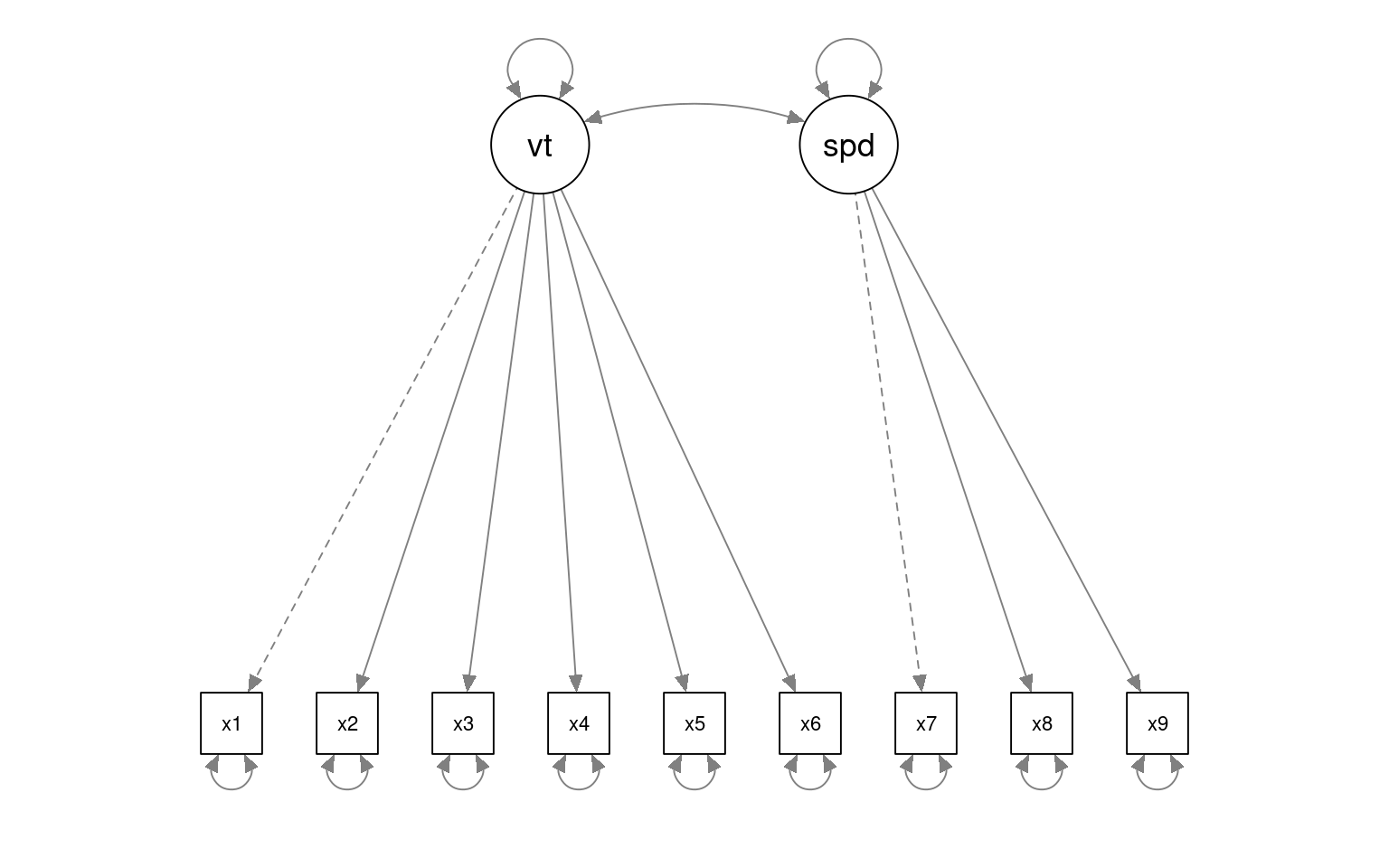These are both ‘significant’ but the test statistic differs a lot. We can test whether they differ significantly using the anova function, which also gives us AIC/BIC statistics.

anova(fit, fit2)
Chi-Squared Difference Test

Df    AIC    BIC   Chisq Chisq diff Df diff Pr(>Chisq)
fit  24 7517.5 7595.3  85.305
fit2 26 7609.5 7680.0 181.337     96.031       2  < 2.2e-16 ***
---
Signif. codes:  0 '***' 0.001 '**' 0.01 '*' 0.05 '.' 0.1 ' ' 1
summary(fit, fit.measures = T)
lavaan 0.6-8 ended normally after 38 iterations

Estimator                                         ML
Optimization method                           NLMINB
Number of model parameters                        21

Number of observations                           301

Model Test User Model:

Test statistic                                85.306
Degrees of freedom                                24
P-value (Chi-square)                           0.000

Model Test Baseline Model:

Test statistic                               918.852
Degrees of freedom                                36
P-value                                        0.000

User Model versus Baseline Model:

Comparative Fit Index (CFI)                    0.931
Tucker-Lewis Index (TLI)                       0.896

Loglikelihood and Information Criteria:

Loglikelihood user model (H0)              -3737.745
Loglikelihood unrestricted model (H1)      -3695.092

Akaike (AIC)                                7517.490
Bayesian (BIC)                              7595.339

Root Mean Square Error of Approximation:

RMSEA                                          0.092
90 Percent confidence interval - lower         0.071
90 Percent confidence interval - upper         0.114
P-value RMSEA <= 0.05                          0.001

Standardized Root Mean Square Residual:

SRMR                                           0.065

Parameter Estimates:

Standard errors                             Standard
Information                                 Expected
Information saturated (h1) model          Structured

Latent Variables:
Estimate  Std.Err  z-value  P(>|z|)
visual =~
x2                1.000
x1                1.807    0.325    5.554    0.000
x3                1.318    0.239    5.509    0.000
textual =~
x4                1.000
x5                1.113    0.065   17.014    0.000
x6                0.926    0.055   16.703    0.000
speed =~
x7                1.000
x8                1.180    0.165    7.152    0.000
x9                1.082    0.151    7.155    0.000

Covariances:
Estimate  Std.Err  z-value  P(>|z|)
visual ~~
textual           0.226    0.052    4.329    0.000
speed             0.145    0.038    3.864    0.000
textual ~~
speed             0.173    0.049    3.518    0.000

Variances:
Estimate  Std.Err  z-value  P(>|z|)
.x2                1.134    0.102   11.146    0.000
.x1                0.549    0.114    4.833    0.000
.x3                0.844    0.091    9.317    0.000
.x4                0.371    0.048    7.778    0.000
.x5                0.446    0.058    7.642    0.000
.x6                0.356    0.043    8.277    0.000
.x7                0.799    0.081    9.823    0.000
.x8                0.488    0.074    6.573    0.000
.x9                0.566    0.071    8.003    0.000
visual            0.248    0.077    3.214    0.001
textual           0.979    0.112    8.737    0.000
speed             0.384    0.086    4.451    0.000
summary(fit2, fit.measures = T)
lavaan 0.6-8 ended normally after 35 iterations

Estimator                                         ML
Optimization method                           NLMINB
Number of model parameters                        19

Number of observations                           301

Model Test User Model:

Test statistic                               181.337
Degrees of freedom                                26
P-value (Chi-square)                           0.000

Model Test Baseline Model:

Test statistic                               918.852
Degrees of freedom                                36
P-value                                        0.000

User Model versus Baseline Model:

Comparative Fit Index (CFI)                    0.824
Tucker-Lewis Index (TLI)                       0.756

Loglikelihood and Information Criteria:

Loglikelihood user model (H0)              -3785.760
Loglikelihood unrestricted model (H1)      -3695.092

Akaike (AIC)                                7609.521
Bayesian (BIC)                              7679.956

Root Mean Square Error of Approximation:

RMSEA                                          0.141
90 Percent confidence interval - lower         0.122
90 Percent confidence interval - upper         0.161
P-value RMSEA <= 0.05                          0.000

Standardized Root Mean Square Residual:

SRMR                                           0.113

Parameter Estimates:

Standard errors                             Standard
Information                                 Expected
Information saturated (h1) model          Structured

Latent Variables:
Estimate  Std.Err  z-value  P(>|z|)
vt =~
x1                1.000
x2                0.509    0.156    3.254    0.001
x3                0.477    0.150    3.189    0.001
x4                1.992    0.272    7.314    0.000
x5                2.194    0.300    7.303    0.000
x6                1.852    0.254    7.294    0.000
speed =~
x7                1.000
x8                1.148    0.163    7.054    0.000
x9                0.891    0.124    7.189    0.000

Covariances:
Estimate  Std.Err  z-value  P(>|z|)
vt ~~
speed             0.095    0.029    3.287    0.001

Variances:
Estimate  Std.Err  z-value  P(>|z|)
.x1                1.112    0.093   11.922    0.000
.x2                1.318    0.108   12.193    0.000
.x3                1.219    0.100   12.196    0.000
.x4                0.373    0.047    7.853    0.000
.x5                0.474    0.059    8.059    0.000
.x6                0.351    0.043    8.221    0.000
.x7                0.732    0.083    8.821    0.000
.x8                0.428    0.082    5.201    0.000
.x9                0.658    0.071    9.300    0.000
vt                0.246    0.067    3.661    0.000
speed             0.451    0.095    4.733    0.000
diagram(fit)
diagram(fit2)

This suggests that the smaller model is fit more poorly according to RMSEA and TLI measures. Also, BIC is 7620 for the smaller model vs 7628 for the larger model, as are the AIC and related measures. Finally, anova chi-squared test is significantly different, indicating the larger model (fit) accounts for the data better than the smaller model (fit2). Altogether, this justifies the 3-factor model over a 2-factor model.

What if we give it a model we don’t think is correct–here f2 is cobbled together:

HS.model3 <- " f1  =~ x1 + x2
f2 =~ x3 + x4
f3 =~ x5 + x6
f4   =~ x7 + x8 + x9 "
fit3 <- cfa(HS.model3, data = HolzingerSwineford1939)
fit3
lavaan 0.6-8 ended normally after 60 iterations

Estimator                                         ML
Optimization method                           NLMINB
Number of model parameters                        24

Number of observations                           301

Model Test User Model:

Test statistic                               144.108
Degrees of freedom                                21
P-value (Chi-square)                           0.000

There is a warning, but it does produce a result. We can use an anova function to compare the two:

anova(fit, fit3)
Chi-Squared Difference Test

Df    AIC    BIC   Chisq Chisq diff Df diff Pr(>Chisq)
fit3 21 7582.3 7671.3 144.108
fit  24 7517.5 7595.3  85.305    -58.803       3          1
# semPlot::semPaths(fit3,title=F,curvePilot=T)
lavaan.diagram(fit3, cut = 0.2, digits = 2)
semPaths(fit3, "par", weighted = TRUE, nCharNodes = 7, shapeMan = "square", sizeMan = 8,
sizeMan2 = 5, layout = "tree2")

Now, there new more complex model, which has more parameters and fewer DF,fits more poorly than the smaller model, so we would again prefer the 3-parameter model.

# The Bifactor model

The bifactor model describes a model where everything is related to one general factor (g), but there are several other specific factors other things are related to.

## Compare to the following model, which has the same structure but it does not
## have the covariance restrictions
HS.modelG <- "G =~ x1 + x2 + x3 + x4 + x5 + x6 + x7  + x8 + x9
visual   =~ x1 + x2 + x3
textual =~ x4 + x5 + x6
speed   =~ x7 + x8 + x9 "

fitG <- cfa(HS.modelG, data = HolzingerSwineford1939)
summary(fitG, fit.measures = T)
lavaan 0.6-8 ended normally after 66 iterations

Estimator                                         ML
Optimization method                           NLMINB
Number of model parameters                        33

Number of observations                           301

Model Test User Model:

Test statistic                                26.020
Degrees of freedom                                12
P-value (Chi-square)                           0.011

Model Test Baseline Model:

Test statistic                               918.852
Degrees of freedom                                36
P-value                                        0.000

User Model versus Baseline Model:

Comparative Fit Index (CFI)                    0.984
Tucker-Lewis Index (TLI)                       0.952

Loglikelihood and Information Criteria:

Loglikelihood user model (H0)              -3708.102
Loglikelihood unrestricted model (H1)      -3695.092

Akaike (AIC)                                7482.204
Bayesian (BIC)                              7604.539

Root Mean Square Error of Approximation:

RMSEA                                          0.062
90 Percent confidence interval - lower         0.029
90 Percent confidence interval - upper         0.095
P-value RMSEA <= 0.05                          0.239

Standardized Root Mean Square Residual:

SRMR                                           0.028

Parameter Estimates:

Standard errors                             Standard
Information                                 Expected
Information saturated (h1) model          Structured

Latent Variables:
Estimate  Std.Err  z-value  P(>|z|)
G =~
x1                1.000
x2                0.613       NA
x3                0.768       NA
x4                0.583       NA
x5                0.552       NA
x6                0.561       NA
x7                0.442       NA
x8                0.619       NA
x9                0.699       NA
visual =~
x1                1.000
x2                0.152       NA
x3                0.127       NA
textual =~
[ reached getOption("max.print") -- omitted 7 rows ]

Covariances:
Estimate  Std.Err  z-value  P(>|z|)
G ~~
visual           -0.061       NA
textual          -0.334       NA
speed            -0.435       NA
visual ~~
textual           0.234       NA
speed             0.063       NA
textual ~~
speed             0.279       NA

Variances:
Estimate  Std.Err  z-value  P(>|z|)
.x1                1.794       NA
.x2                1.025       NA
.x3                0.683       NA
.x4                0.379       NA
.x5                0.398       NA
.x6                0.368       NA
.x7                0.633       NA
.x8                0.469       NA
.x9                0.575       NA
G                 1.062       NA
visual           -1.377       NA
textual           1.000       NA
speed             0.727       NA                  
HS.model4 <- "g=~ x1 + x2 + x3 + x4 + x5 + x6 + x7 + x8 + x9
f1  =~ x1 + x2 + x3
f2 =~ x4 + x5 + x6
f3   =~ x7 + x8 + x9
g ~~ 0*f1
g ~~ 0*f2
g ~~ 0*f3
f1~~ 0*f1
f2~~ 0*f2
f3 ~~ 0*f3
"

fit4 <- cfa(HS.model4, data = HolzingerSwineford1939)
# fit4 <- cfa(HS.model4,data=HolzingerSwineford1939,estimator='WLS')
fit4
lavaan 0.6-8 ended normally after 48 iterations

Estimator                                         ML
Optimization method                           NLMINB
Number of model parameters                        27

Number of observations                           301

Model Test User Model:

Test statistic                                56.159
Degrees of freedom                                18
P-value (Chi-square)                           0.000
summary(fit4, fit.measures = T)
lavaan 0.6-8 ended normally after 48 iterations

Estimator                                         ML
Optimization method                           NLMINB
Number of model parameters                        27

Number of observations                           301

Model Test User Model:

Test statistic                                56.159
Degrees of freedom                                18
P-value (Chi-square)                           0.000

Model Test Baseline Model:

Test statistic                               918.852
Degrees of freedom                                36
P-value                                        0.000

User Model versus Baseline Model:

Comparative Fit Index (CFI)                    0.957
Tucker-Lewis Index (TLI)                       0.914

Loglikelihood and Information Criteria:

Loglikelihood user model (H0)              -3723.172
Loglikelihood unrestricted model (H1)      -3695.092

Akaike (AIC)                                7500.344
Bayesian (BIC)                              7600.435

Root Mean Square Error of Approximation:

RMSEA                                          0.084
90 Percent confidence interval - lower         0.060
90 Percent confidence interval - upper         0.109
P-value RMSEA <= 0.05                          0.013

Standardized Root Mean Square Residual:

SRMR                                           0.051

Parameter Estimates:

Standard errors                             Standard
Information                                 Expected
Information saturated (h1) model          Structured

Latent Variables:
Estimate  Std.Err  z-value  P(>|z|)
g =~
x1                1.000
x2                0.685    0.121    5.655    0.000
x3                0.921    0.151    6.118    0.000
x4                1.235    0.144    8.579    0.000
x5                1.401    0.163    8.623    0.000
x6                1.140    0.134    8.536    0.000
x7                0.841    0.125    6.746    0.000
x8                0.986    0.133    7.427    0.000
x9                0.725    0.111    6.557    0.000
f1 =~
x1                1.000
x2                0.893    0.238    3.758    0.000
x3                1.205    0.299    4.033    0.000
f2 =~
[ reached getOption("max.print") -- omitted 7 rows ]

Covariances:
Estimate  Std.Err  z-value  P(>|z|)
g ~~
f1                0.000
f2                0.000
f3                0.000
f1 ~~
f2               -0.411    0.084   -4.897    0.000
f3               -0.349    0.081   -4.320    0.000
f2 ~~
f3               -0.523    0.084   -6.225    0.000

Variances:
Estimate  Std.Err  z-value  P(>|z|)
f1                0.000
f2                0.000
f3                0.000
.x1                0.703    0.113    6.252    0.000
.x2                1.070    0.101   10.545    0.000
.x3                0.711    0.102    6.996    0.000
.x4                0.383    0.048    7.939    0.000
.x5                0.416    0.058    7.143    0.000
.x6                0.370    0.044    8.482    0.000
.x7                0.737    0.084    8.751    0.000
.x8                0.406    0.086    4.704    0.000
.x9                0.680    0.071    9.558    0.000
g                 0.640    0.135    4.748    0.000
semPaths(fit4, "par", weighted = FALSE, nCharNodes = 7, shapeMan = "rectangle", sizeMan = 8,
sizeMan2 = 5)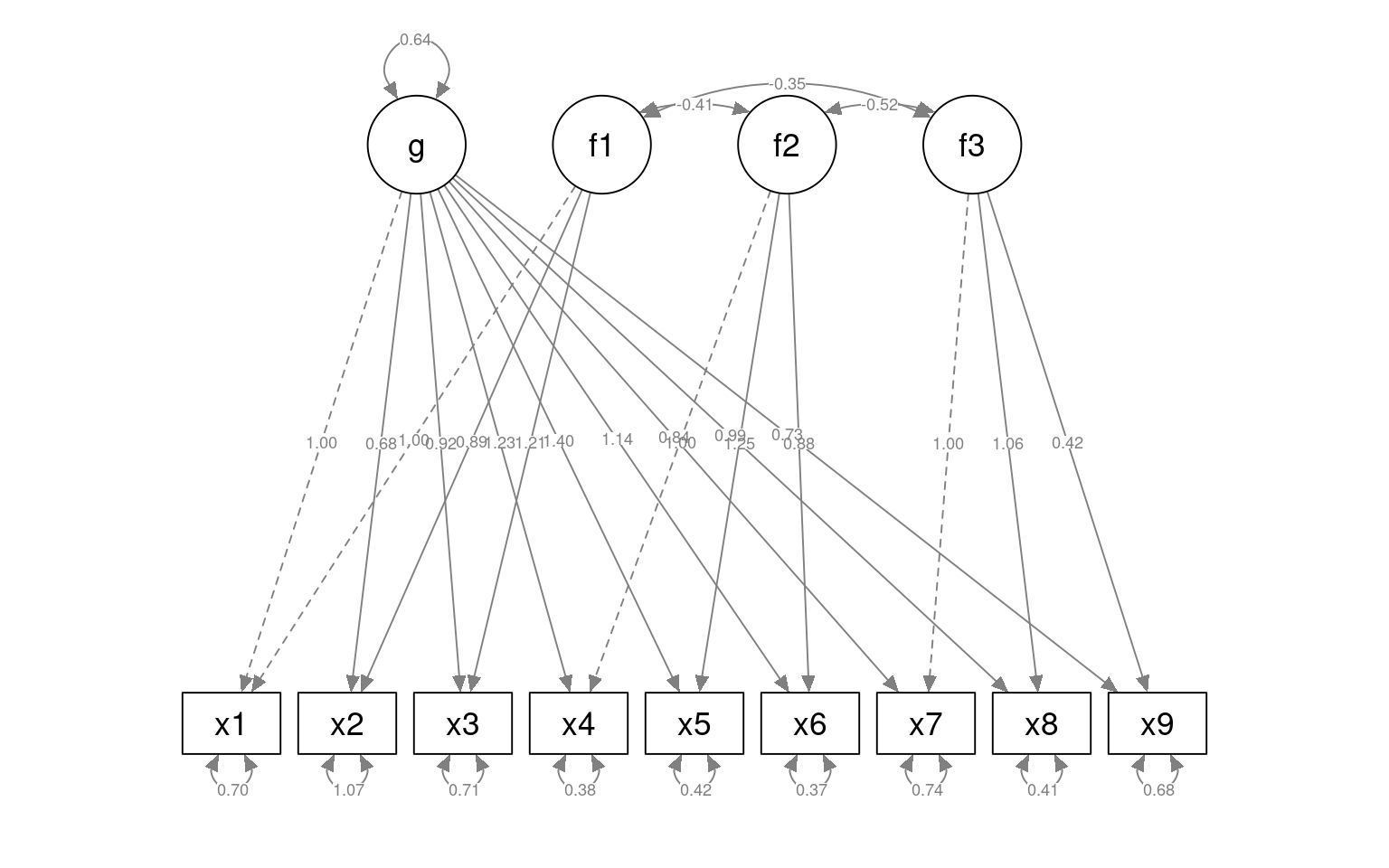HS.model4b <- "g=~ x1 + x2 + x3 + x4 + x5 + x6 + x7 + x8 + x9
f1  =~ x1 + x2 + x3
f2 =~ x4 + x5 + x6
f3   =~ x7 + x8
g ~~ 0*f1
g ~~ 0*f2
g ~~ 0*f3
f1~~ 0*f1
f2~~ 0*f2
f3 ~~ 0*f3
"
fit4b <- cfa(HS.model4b, data = HolzingerSwineford1939)
summary(fit4b, fit.measures = T)
lavaan 0.6-8 ended normally after 44 iterations

Estimator                                         ML
Optimization method                           NLMINB
Number of model parameters                        26

Number of observations                           301

Model Test User Model:

Test statistic                                65.101
Degrees of freedom                                19
P-value (Chi-square)                           0.000

Model Test Baseline Model:

Test statistic                               918.852
Degrees of freedom                                36
P-value                                        0.000

User Model versus Baseline Model:

Comparative Fit Index (CFI)                    0.948
Tucker-Lewis Index (TLI)                       0.901

Loglikelihood and Information Criteria:

Loglikelihood user model (H0)              -3727.643
Loglikelihood unrestricted model (H1)      -3695.092

Akaike (AIC)                                7507.285
Bayesian (BIC)                              7603.670

Root Mean Square Error of Approximation:

RMSEA                                          0.090
90 Percent confidence interval - lower         0.066
90 Percent confidence interval - upper         0.114
P-value RMSEA <= 0.05                          0.003

Standardized Root Mean Square Residual:

SRMR                                           0.063

Parameter Estimates:

Standard errors                             Standard
Information                                 Expected
Information saturated (h1) model          Structured

Latent Variables:
Estimate  Std.Err  z-value  P(>|z|)
g =~
x1                1.000
x2                0.626    0.106    5.902    0.000
x3                0.822    0.119    6.913    0.000
x4                1.173    0.122    9.591    0.000
x5                1.340    0.139    9.632    0.000
x6                1.085    0.114    9.538    0.000
x7                0.784    0.116    6.742    0.000
x8                0.932    0.127    7.363    0.000
x9                0.507    0.068    7.429    0.000
f1 =~
x1                1.000
x2                0.747    0.166    4.512    0.000
x3                0.986    0.185    5.335    0.000
f2 =~
[ reached getOption("max.print") -- omitted 6 rows ]

Covariances:
Estimate  Std.Err  z-value  P(>|z|)
g ~~
f1                0.000
f2                0.000
f3                0.000
f1 ~~
f2               -0.434    0.075   -5.808    0.000
f3               -0.457    0.088   -5.171    0.000
f2 ~~
f3               -0.533    0.085   -6.237    0.000

Variances:
Estimate  Std.Err  z-value  P(>|z|)
f1                0.000
f2                0.000
f3                0.000
.x1                0.618    0.105    5.867    0.000
.x2                1.091    0.100   10.855    0.000
.x3                0.773    0.091    8.450    0.000
.x4                0.387    0.048    8.063    0.000
.x5                0.419    0.058    7.224    0.000
.x6                0.370    0.043    8.546    0.000
.x7                0.723    0.096    7.563    0.000
.x8                0.375    0.107    3.500    0.000
.x9                0.827    0.062   13.266    0.000
g                 0.734    0.136    5.414    0.000
anova(fit4, fit4b)
Chi-Squared Difference Test

Df    AIC    BIC  Chisq Chisq diff Df diff Pr(>Chisq)
fit4  18 7500.3 7600.4 56.159
fit4b 19 7507.3 7603.7 65.101     8.9415       1   0.002788 **
---
Signif. codes:  0 '***' 0.001 '**' 0.01 '*' 0.05 '.' 0.1 ' ' 1
semPaths(fit4, "model", "est", curvePivot = FALSE, edge.label.cex = 0.5)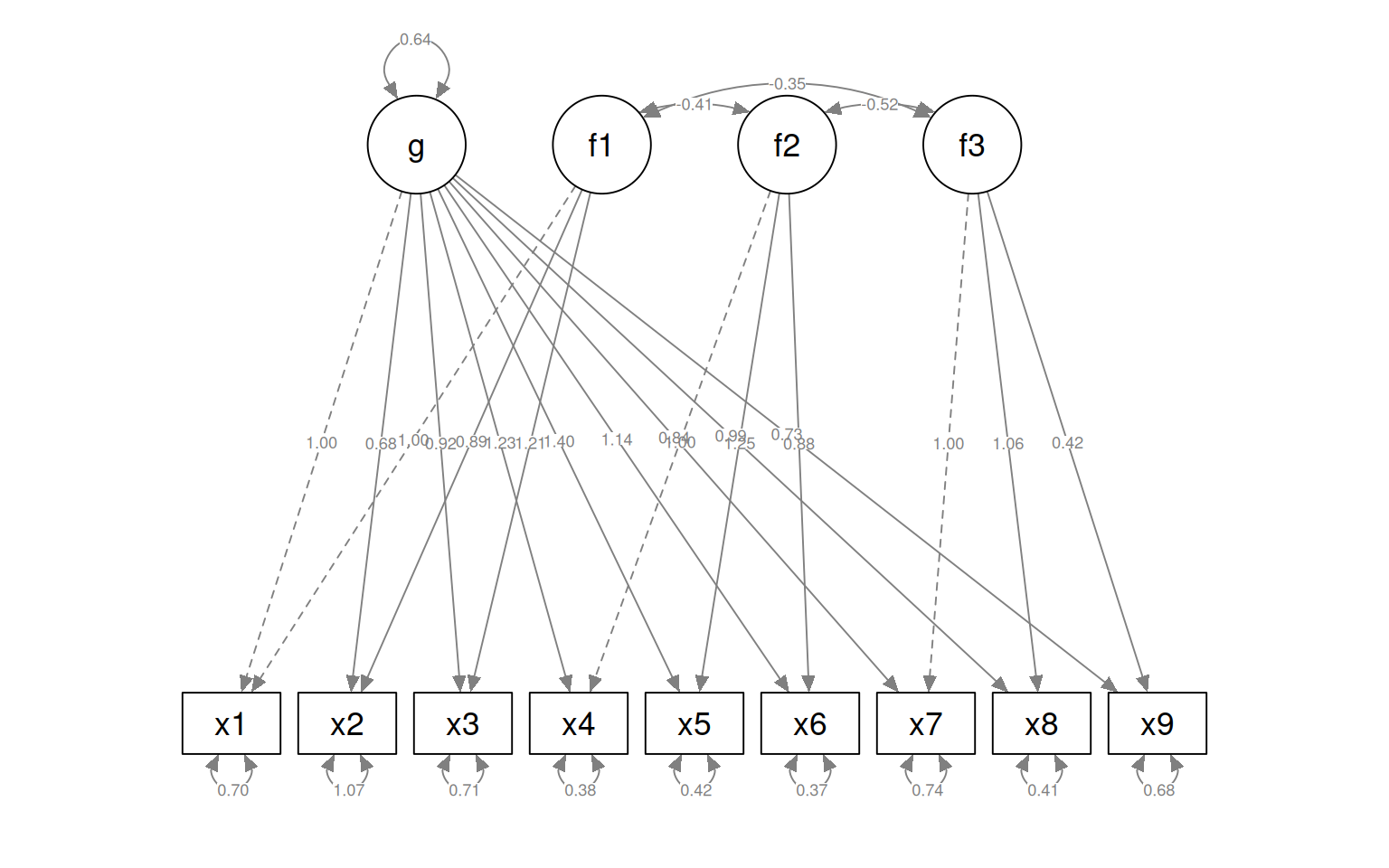semPaths(fit4, "model", "est", layout = "circle", curvePivot = FALSE, edge.label.cex = 0.5)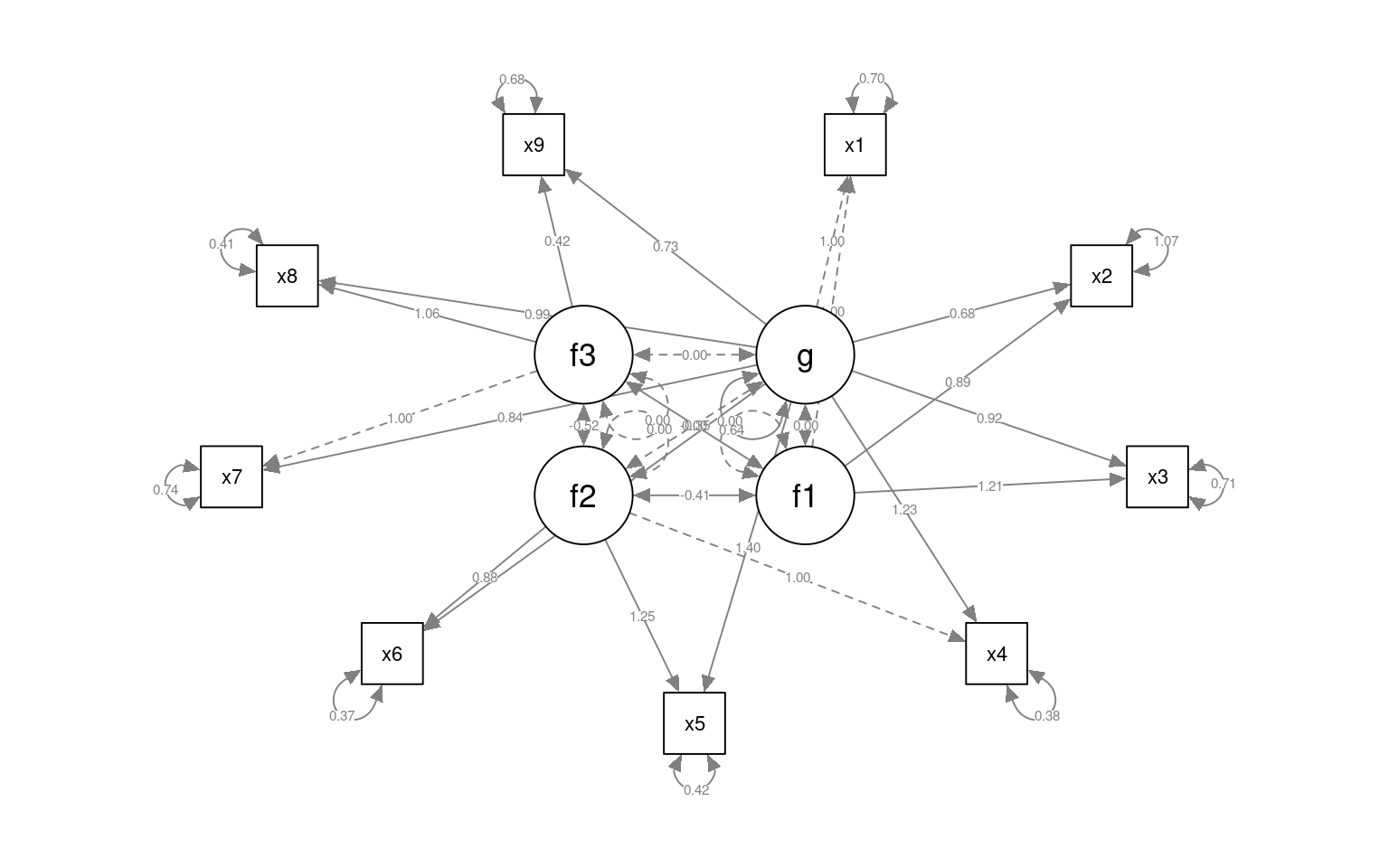semPaths(fit4, "model", "est", layout = "tree", curvePivot = FALSE, edge.label.cex = 0.5)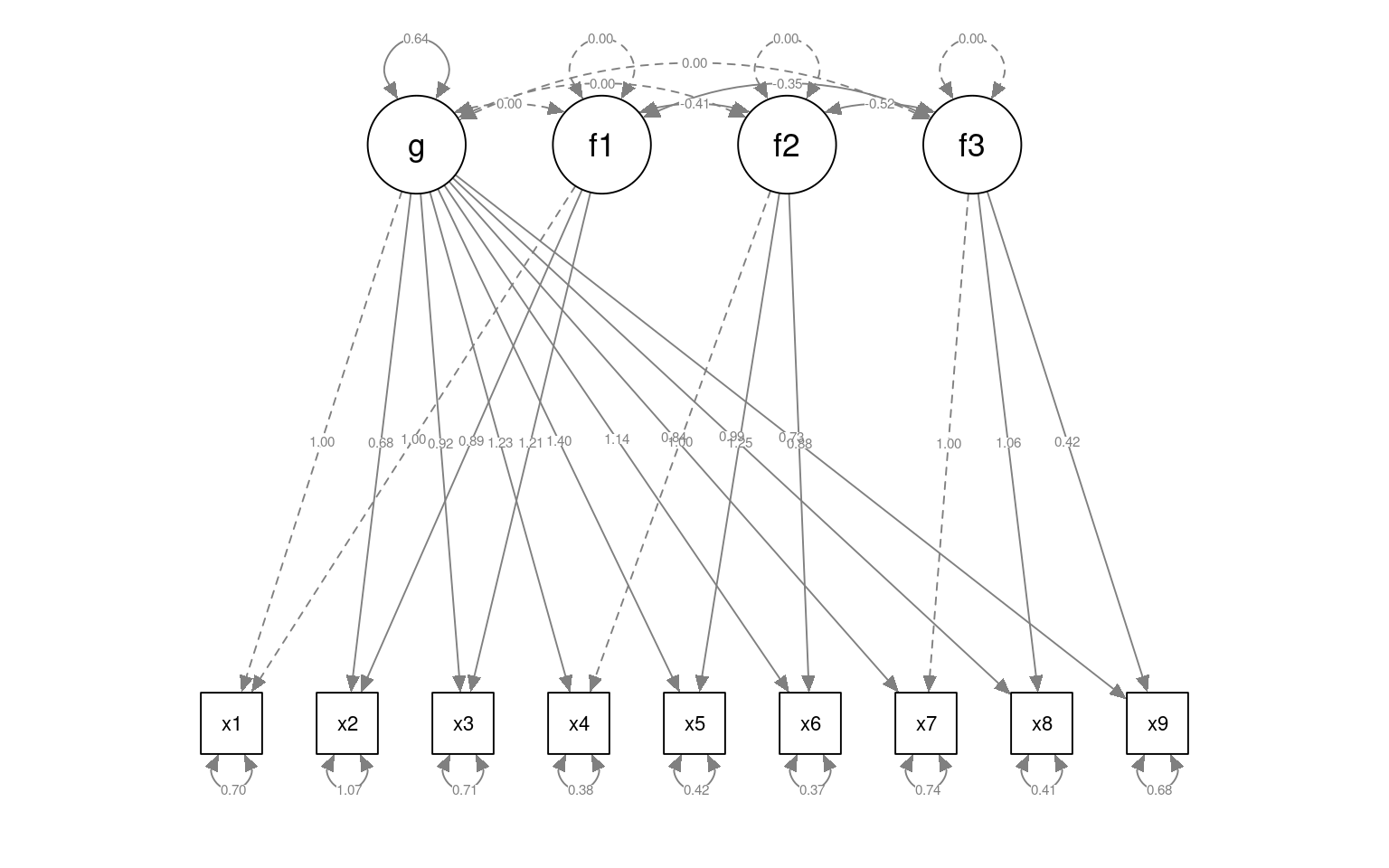lavaan.diagram(fit4, cut = 0.2, digits = 2)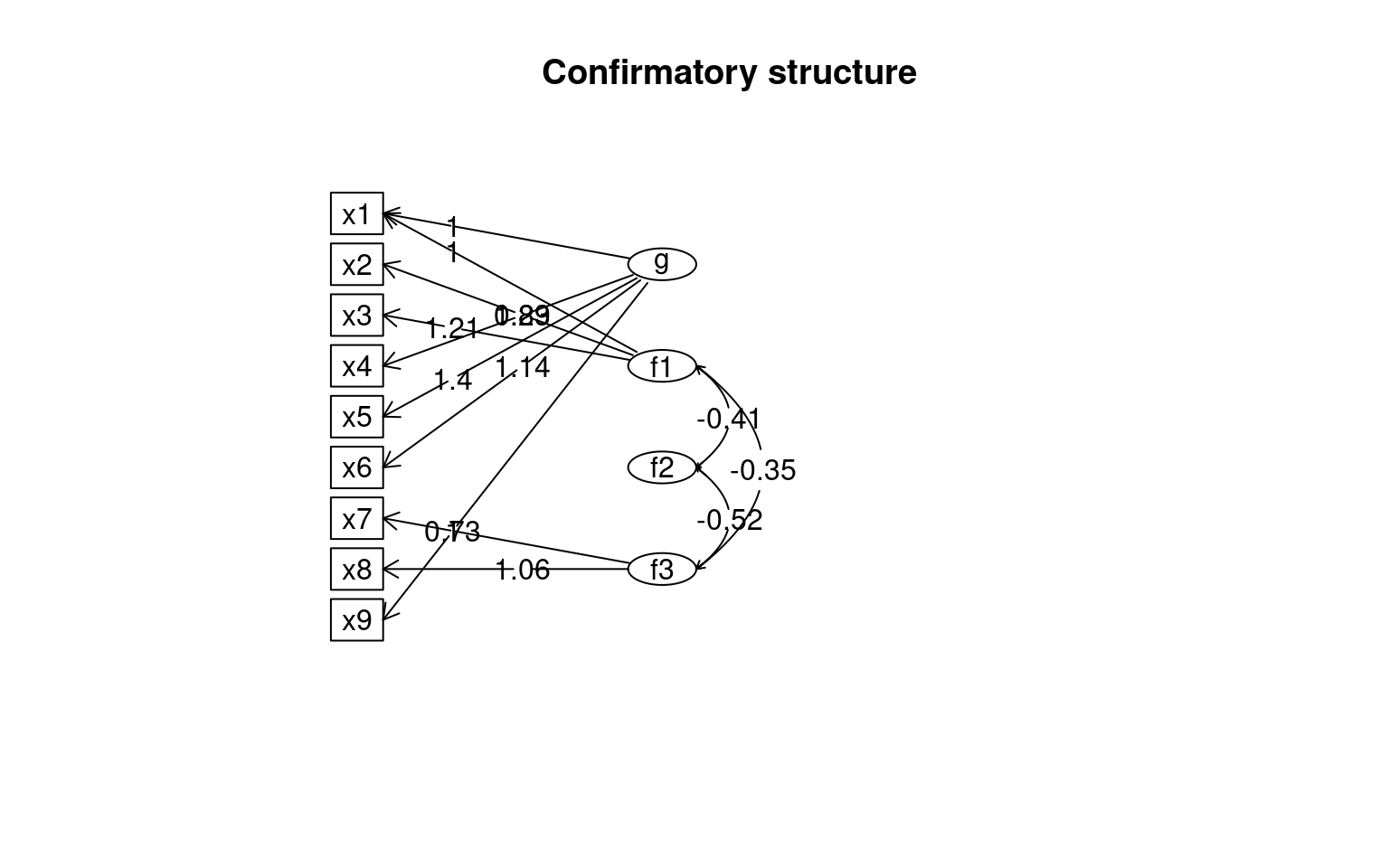semPaths(fit4, "par", weighted = FALSE, nCharNodes = 7, shapeMan = "rectangle", sizeMan = 8,
sizeMan2 = 5)anova(fit4, fit)
Chi-Squared Difference Test

Df    AIC    BIC  Chisq Chisq diff Df diff Pr(>Chisq)
fit4 18 7500.3 7600.4 56.159
fit  24 7517.5 7595.3 85.305     29.146       6  5.708e-05 ***
---
Signif. codes:  0 '***' 0.001 '**' 0.01 '*' 0.05 '.' 0.1 ' ' 1

Here, we have mixed results; a Chi-squared test shows the more complex model is better, as does AIC; but BIC is pessimistic, feeling it is too complex. Looking at the parameter estimates, there are some strange things; we no longer have consistent positive loadings to f1, and we get a warning that it is not positive definite, which should make us wary that our data is not sufficiently constraining our model.

We can force the latent variables to be uncorrelated. In this case, the model does not converge, and so there is not much we can take away from it, but it might be useful in some situations. Plus, below we can see code that let’s us see the residuals of the correlation matrix, the basis for RMSE and RMSEA measures.

fit5 <- cfa(HS.model4, data = HolzingerSwineford1939, orthogonal = TRUE)
summary(fit5, fit.measures = T)
lavaan 0.6-8 ended normally after 31 iterations

Estimator                                         ML
Optimization method                           NLMINB
Number of model parameters                        24

Number of observations                           301

Model Test User Model:

Test statistic                               312.264
Degrees of freedom                                21
P-value (Chi-square)                           0.000

Model Test Baseline Model:

Test statistic                               918.852
Degrees of freedom                                36
P-value                                        0.000

User Model versus Baseline Model:

Comparative Fit Index (CFI)                    0.670
Tucker-Lewis Index (TLI)                       0.434

Loglikelihood and Information Criteria:

Loglikelihood user model (H0)              -3851.224
Loglikelihood unrestricted model (H1)      -3695.092

Akaike (AIC)                                7750.448
Bayesian (BIC)                              7839.419

Root Mean Square Error of Approximation:

RMSEA                                          0.215
90 Percent confidence interval - lower         0.194
90 Percent confidence interval - upper         0.236
P-value RMSEA <= 0.05                          0.000

Standardized Root Mean Square Residual:

SRMR                                           0.143

Parameter Estimates:

Standard errors                             Standard
Information                                 Expected
Information saturated (h1) model          Structured

Latent Variables:
Estimate  Std.Err  z-value  P(>|z|)
g =~
x1                1.000
x2                0.508       NA
x3                0.493       NA
x4                1.930       NA
x5                2.123       NA
x6                1.796       NA
x7                0.385       NA
x8                0.398       NA
x9                0.606       NA
f1 =~
x1                1.000
x2                0.778       NA
x3                1.107       NA
f2 =~
[ reached getOption("max.print") -- omitted 7 rows ]

Covariances:
Estimate  Std.Err  z-value  P(>|z|)
g ~~
f1                0.000
f2                0.000
f3                0.000
f1 ~~
f2                0.000
f3                0.000
f2 ~~
f3                0.000

Variances:
Estimate  Std.Err  z-value  P(>|z|)
f1                0.000
f2                0.000
f3                0.000
.x1                1.098       NA
.x2                1.315       NA
.x3                1.212       NA
.x4                0.380       NA
.x5                0.486       NA
.x6                0.356       NA
.x7                1.145       NA
.x8                0.981       NA
.x9                0.919       NA
g                 0.261       NA                  
semPaths(fit5, "model", "est", curvePivot = FALSE, edge.label.cex = 0.5)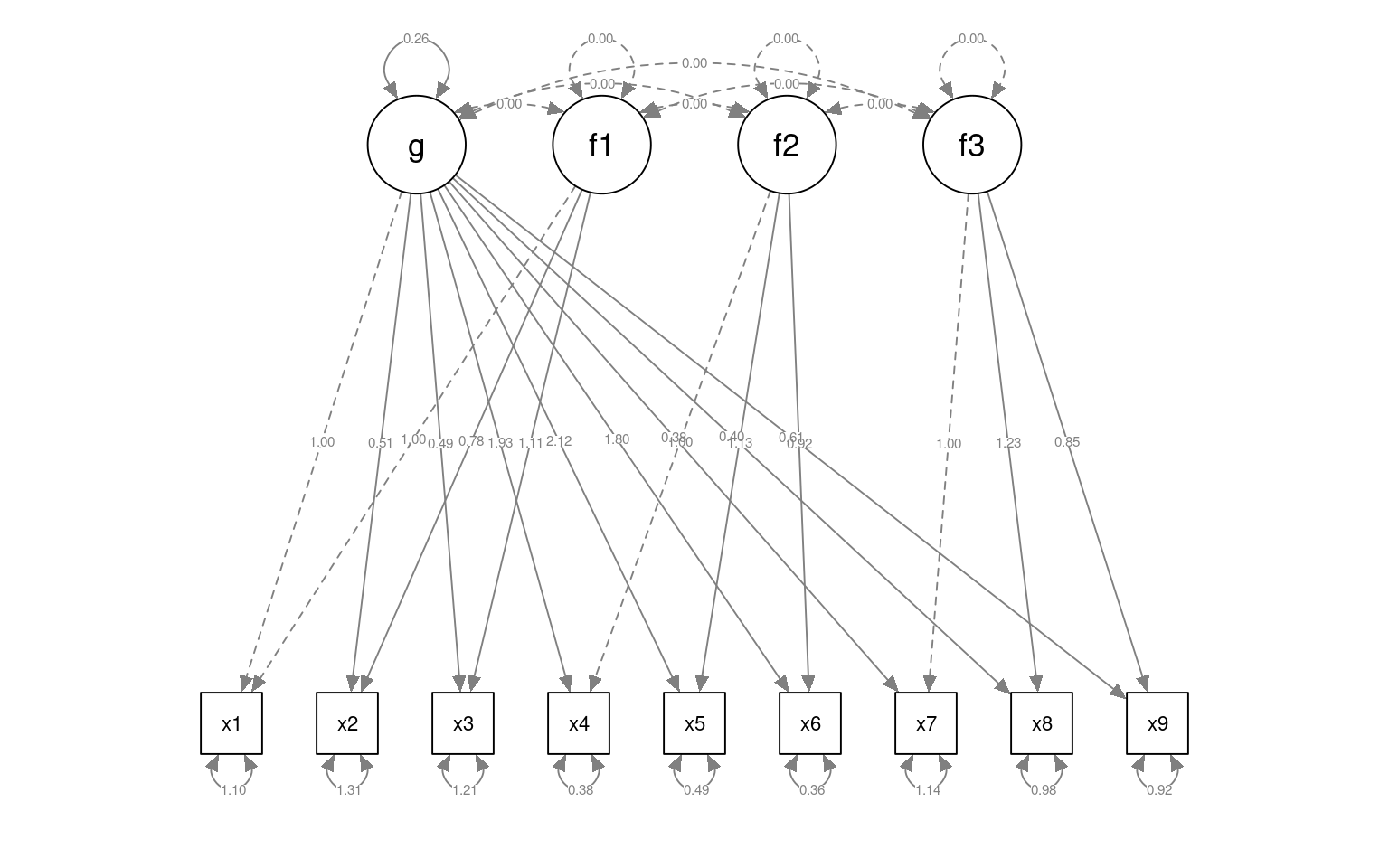residuals(fit5, type = "cor")
$type  "cor.bollen"$cov
x1     x2     x3     x4     x5     x6     x7     x8     x9
x1  0.000
x2  0.201  0.000
x3  0.343  0.291  0.000
x4  0.001 -0.034 -0.030  0.000
x5 -0.075 -0.046 -0.110  0.020  0.000
x6 -0.010  0.008  0.011 -0.006  0.015  0.000
x7 -0.012 -0.115  0.032  0.021 -0.050 -0.030  0.000
x8  0.136  0.048  0.141 -0.064 -0.031 -0.019  0.450  0.000
[ reached getOption("max.print") -- omitted 1 row ]

## Bifactor model with the omega function

A traditional version of the bifactor model is available in the omega function in PSYCH, where the factors are automatically identified. This is more like EFA with a specific structure. Also, we discussed this model when examining psychometrics: this is argued to be the best way to identify whether a single factor exists, and more instructive than measures such as cohen’s or GLB.

library(psych)

holz <- omega(cor(HolzingerSwineford1939[, 7:15]), 3, title = "14 ability tests from Holzinger-Swineford")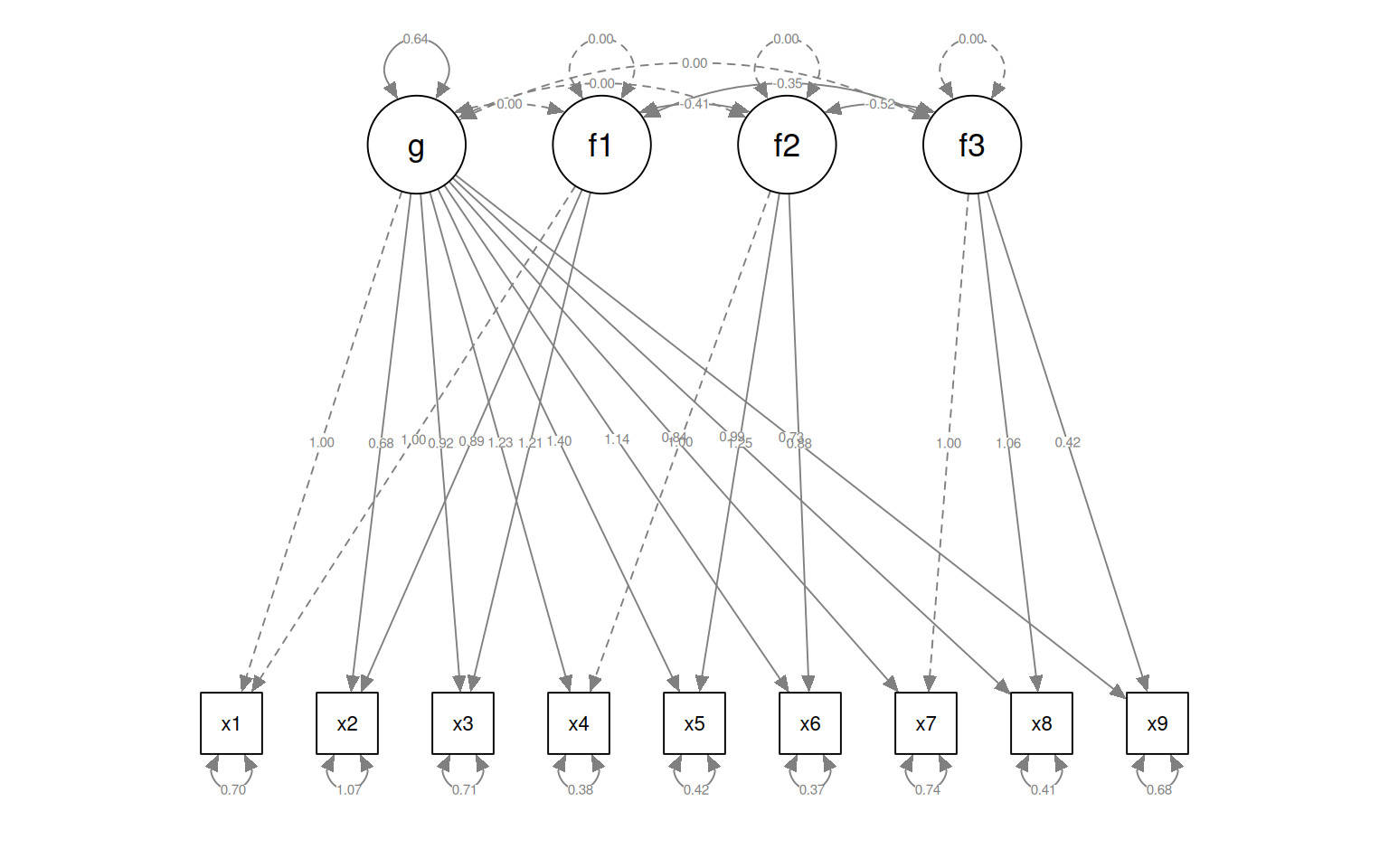holz
14 ability tests from Holzinger-Swineford
Call: omegah(m = m, nfactors = nfactors, fm = fm, key = key, flip = flip,
digits = digits, title = title, sl = sl, labels = labels,
plot = plot, n.obs = n.obs, rotate = rotate, Phi = Phi, option = option,
covar = covar)
Alpha:                 0.76
G.6:                   0.81
Omega Hierarchical:    0.45
Omega H asymptotic:    0.53
Omega Total            0.85

g   F1*   F2*   F3*   h2   u2   p2
x1 0.49        0.46       0.48 0.52 0.49
x2 0.29        0.40       0.26 0.74 0.33
x3 0.41        0.53       0.45 0.55 0.37
x4 0.45  0.73             0.73 0.27 0.28
x5 0.42  0.76             0.75 0.25 0.23
x6 0.46  0.69             0.69 0.31 0.30
x7 0.23              0.67 0.52 0.48 0.10
x8 0.35              0.62 0.52 0.48 0.23
x9 0.45        0.30  0.42 0.46 0.54 0.43

With eigenvalues of:
g  F1*  F2*  F3*
1.44 1.62 0.77 1.03

general/max  0.89   max/min =   2.09
mean percent general =  0.31    with sd =  0.12 and cv of  0.38
Explained Common Variance of the general factor =  0.3

The degrees of freedom are 12  and the fit is  0.08

The root mean square of the residuals is  0.02
The df corrected root mean square of the residuals is  0.03

Compare this with the adequacy of just a general factor and no group factors
The degrees of freedom for just the general factor are 27  and the fit is  1.75

The root mean square of the residuals is  0.2
The df corrected root mean square of the residuals is  0.23

g  F1*   F2*  F3*
Correlation of scores with factors             0.68 0.84  0.67 0.79
Multiple R square of scores with factors       0.46 0.71  0.45 0.62
Minimum correlation of factor score estimates -0.08 0.42 -0.10 0.23

Total, General and Subset omega for each subset
g  F1*  F2*  F3*
Omega total for total scores and subscales    0.85 0.89 0.65 0.72
Omega general for total scores and subscales  0.45 0.24 0.27 0.19
Omega group for total scores and subscales    0.35 0.65 0.37 0.53

# More on the lavaan syntax and operators

The lavaan syntax lets you describe models with 4 operators:

• ~ Regression (structural model)
• =~ Latent variable definition (also measurement model)
• ~~ Specifying and fixing residual correlations and covariances
• ~1 Specifying intercepts

The third operator ~~ is tricky to understand. The most common use for this is that you are essentially adding the possibility for measured variables to be correlated (vs uncorrelated), or forcing them to be independent, capturing something not otherwise captured by latent variables. You can also use coefficient notation to force covariances to be of some value (helpful because the first covariance is set to 1.0 in a latent model) For example, you might add

  L1 ~~ 0*L2

to force independence of latent variables L1 and L2.

or

  L1 =~ f1 + 1*f2 + f3

The ~1 operator is useful for regression-style modeling, which is used for what are called latent growth models, used for longitidinal, aging, and development research.

# More complex models

Confirmatory factor analysis typically identifies a single set of factors and tries to model the data in that way. But the lavaan library offers more complex structural equation modeling and latent growth curve modeling, and general latent variable regressions, which is also useful in complex situations. For example, you could combine several measures to create a factor, which you then use as a regression predictor or outcome variable to establish relationships between hidden/latent variables. In developmental circles, it is common to use latent growth models to model the development or decline of specific latent cognitive or social behaviors or skills.

A common type of analysis that lavaan permits is looking at the role of mediating variables. There are many tools available for specifically looking at 3-variable problems, but lavaan lets you model arbitrarily complex mediation schemes. The analysis of these is more ad hoc though.

## Mediation Analysis

The notion of doing a mediation analysis is central to many areas of psychology, especially in social domains where you cannot have complete experimental control. In these cases, you will always have potentially confounding variables, and mediation analysis examines a particular kind of causal relationship. Some of the papers describing mediation are among the top 20 scientific research papers cited in any domain.

The notion is that you may observe a correlation between two variables, but also observe that these may be correlated with a third. For example, you might find that as a population ages from teenager to adult, vehicular accidents go down. But, it might not be age per se that causes this. Instead, it might be practice…as you get older, you get more experience driving, and the experience reduces accidents. Experience may be a mediating variable here. Can all the improvement in driver safety be attributed to experience? Maybe, but to find out, you need to study people who started driving at different ages. This would be a classic mediation test.

Below is an example of how we would model this with lavaan. Here, using standard terminology, y is the outcome (accident rate), X is the predictor (age), and M is the mediator variable (experience).

set.seed(1234)

X <- rnorm(100)
M <- 0.5 * X + rnorm(100)
Y <- 0.7 * M + rnorm(100)

M2 <- 0.6 * X + rnorm(100)
Y2 <- 0.6 * X + rnorm(100)

Data <- data.frame(X = X, Y = Y, M = M)
Data2 <- data.frame(X = X, Y = Y2, M = M2)

pairs(Data)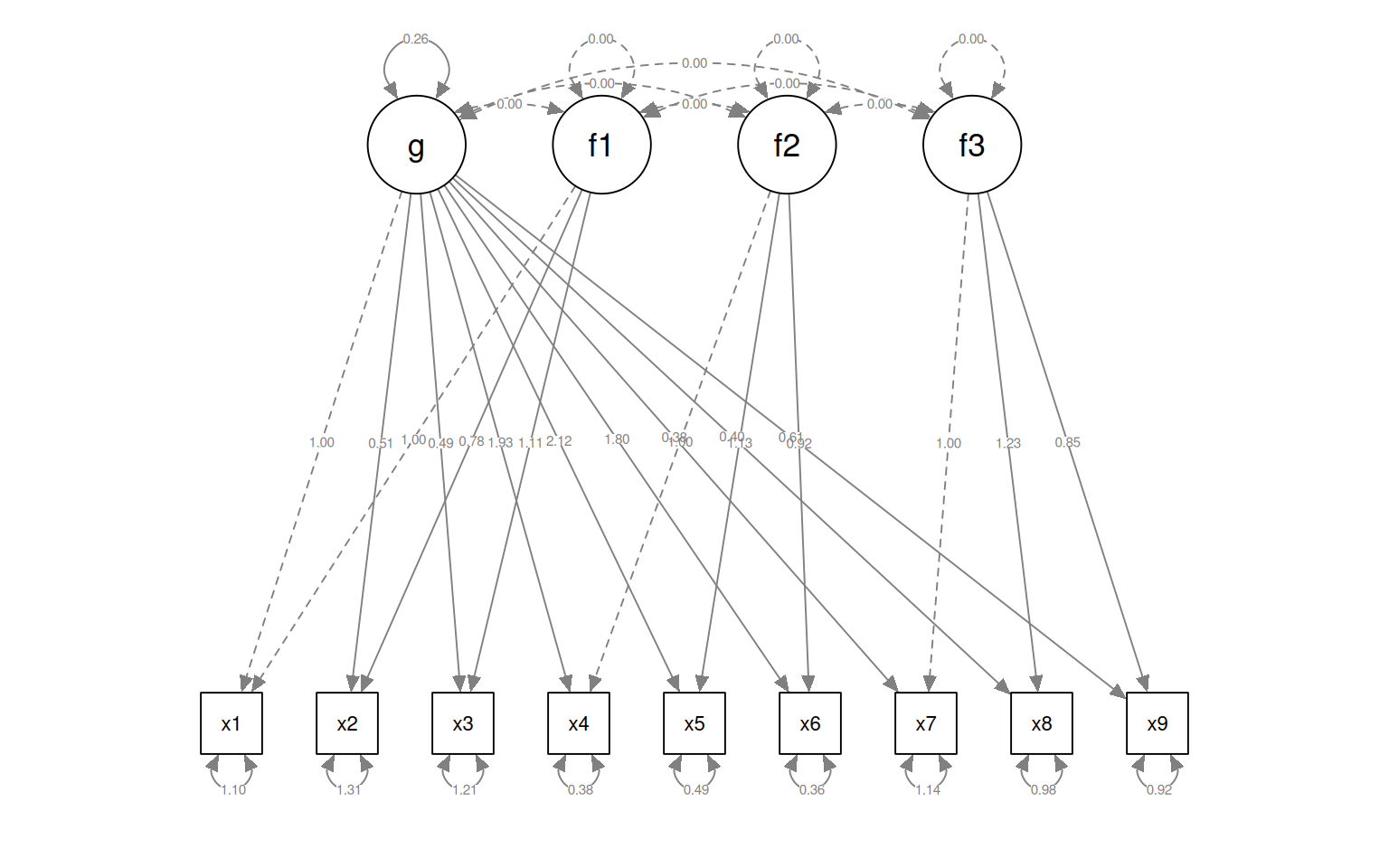cor(Data)
          X         Y         M
X 1.0000000 0.3122748 0.4188856
Y 0.3122748 1.0000000 0.6909791
M 0.4188856 0.6909791 1.0000000
pairs(Data2)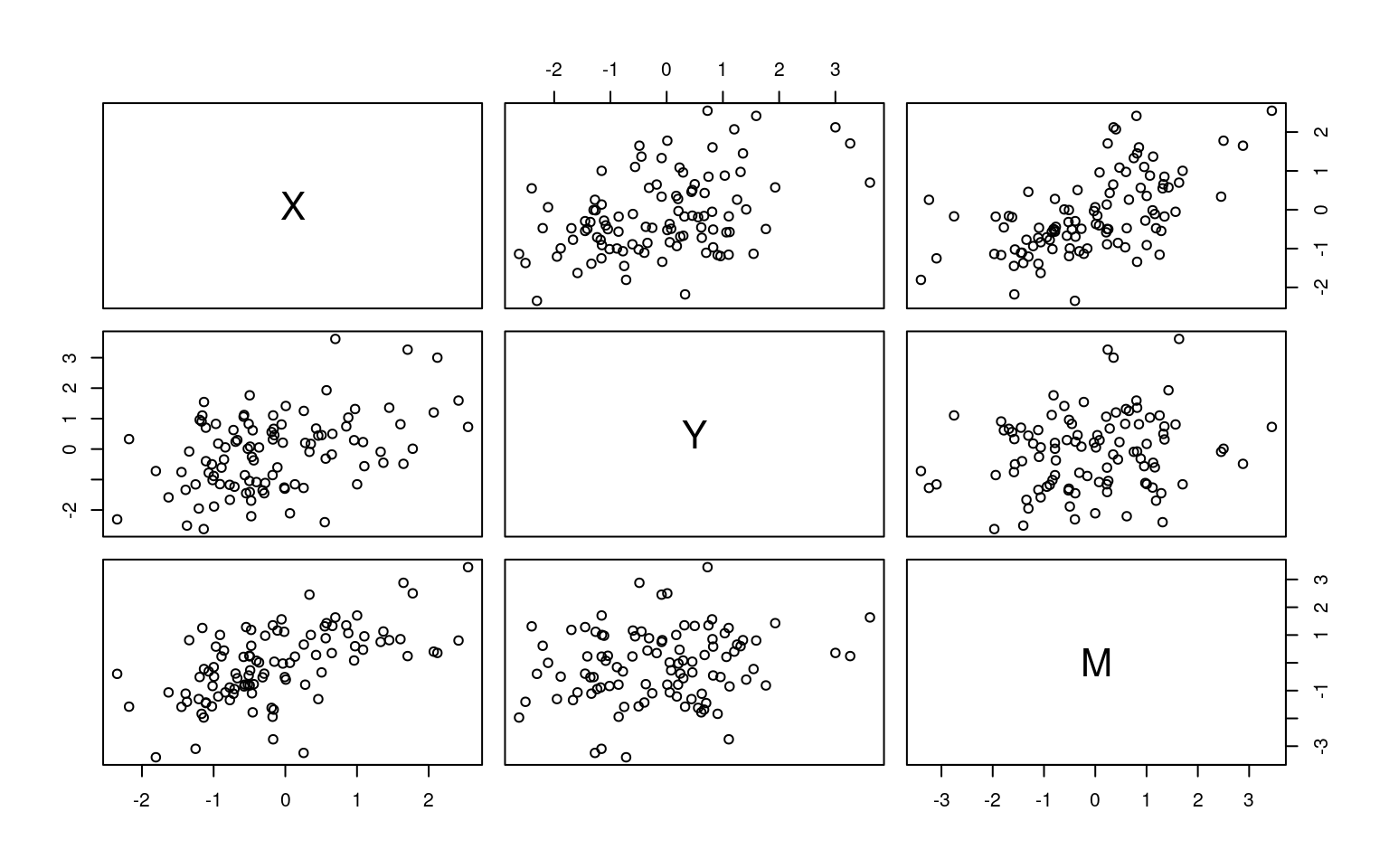cor(Data2)
          X         Y         M
X 1.0000000 0.4255321 0.5857204
Y 0.4255321 1.0000000 0.1827901
M 0.5857204 0.1827901 1.0000000

So, for both Data and Data2, we have three variables that are all correlated. In truth, for Data, Y is related only to M, but M is related to X. For Data2 both M and Y are related to X directly.

We use ~ to model direct relationships between observed variables. Here, we will fit a model with a direct relationship between X and Y, and also another link through M. We need to specify a variable to represent the interaction of a and b, using :=, and a final variance total that captures everthing.

library(lavaan)

library(semPlot)

model <- " # direct effect
Y ~ c*X
# mediator
M ~ a*X
Y ~ b*M
# indirect effect (a*b)
ab := a*b
# total effect
total := c + (a*b)
"
fitmed <- sem(model, data = Data)
summary(fitmed)
lavaan 0.6-8 ended normally after 12 iterations

Estimator                                         ML
Optimization method                           NLMINB
Number of model parameters                         5

Number of observations                           100

Model Test User Model:

Test statistic                                 0.000
Degrees of freedom                                 0

Parameter Estimates:

Standard errors                             Standard
Information                                 Expected
Information saturated (h1) model          Structured

Regressions:
Estimate  Std.Err  z-value  P(>|z|)
Y ~
X          (c)    0.036    0.104    0.348    0.728
M ~
X          (a)    0.474    0.103    4.613    0.000
Y ~
M          (b)    0.788    0.092    8.539    0.000

Variances:
Estimate  Std.Err  z-value  P(>|z|)
.Y                 0.898    0.127    7.071    0.000
.M                 1.054    0.149    7.071    0.000

Defined Parameters:
Estimate  Std.Err  z-value  P(>|z|)
ab                0.374    0.092    4.059    0.000
total             0.410    0.125    3.287    0.001
semPaths(fitmed, title = F, curvePilot = T, "par", weighted = FALSE, nCharNodes = 7,
shapeMan = "rectangle", sizeMan = 15, sizeMan2 = 10)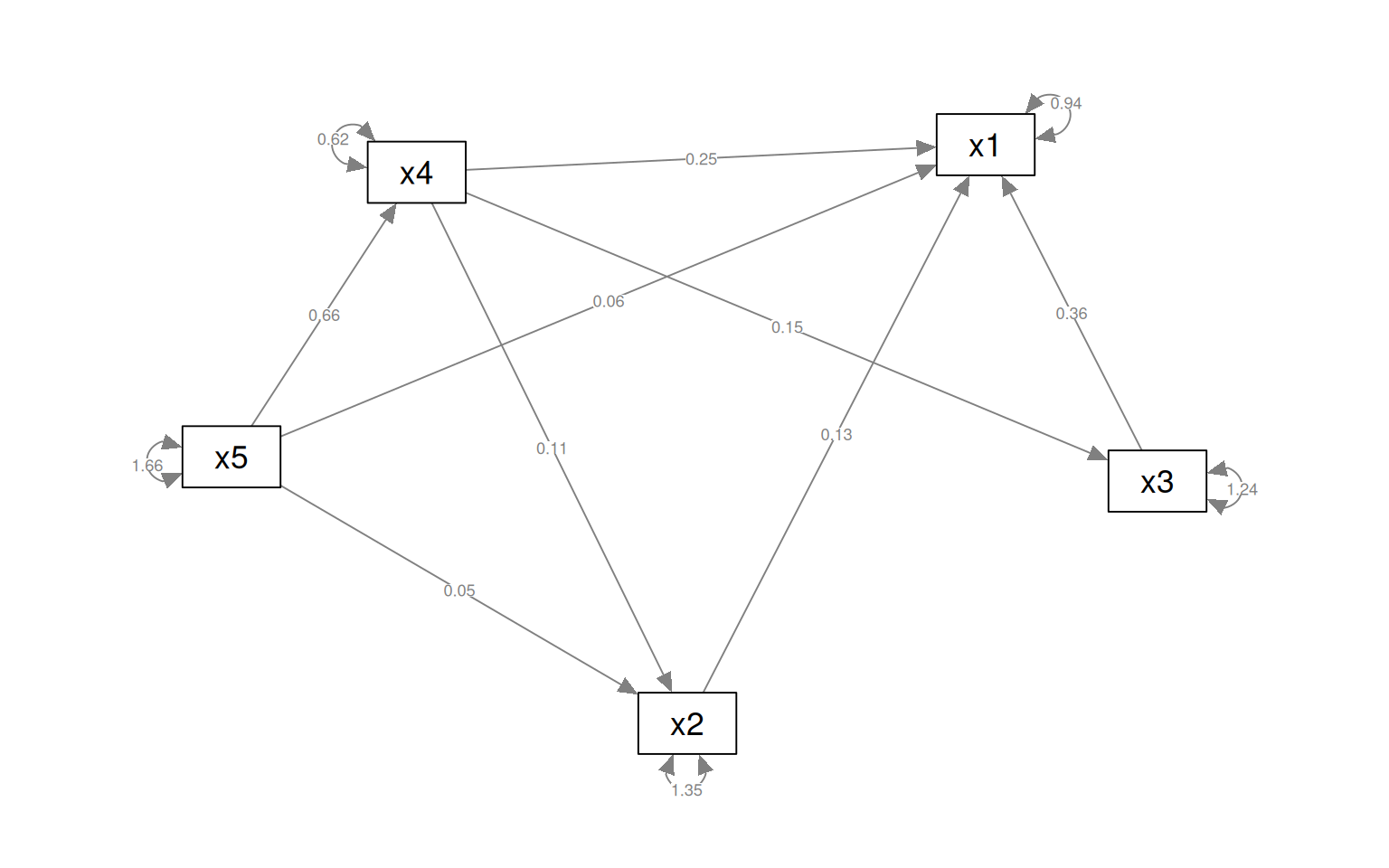Looking at this first model (where M really mediates X-Y), we see that the edges X-M and M-Y are strong, but X->Y is weak, indicating M mediates the relationship between X and Y.

fitmed2 <- sem(model, data = Data2)
summary(fitmed2)
lavaan 0.6-8 ended normally after 15 iterations

Estimator                                         ML
Optimization method                           NLMINB
Number of model parameters                         5

Number of observations                           100

Model Test User Model:

Test statistic                                 0.000
Degrees of freedom                                 0

Parameter Estimates:

Standard errors                             Standard
Information                                 Expected
Information saturated (h1) model          Structured

Regressions:
Estimate  Std.Err  z-value  P(>|z|)
Y ~
X          (c)    0.594    0.136    4.360    0.000
M ~
X          (a)    0.748    0.104    7.227    0.000
Y ~
M          (b)   -0.097    0.107   -0.910    0.363

Variances:
Estimate  Std.Err  z-value  P(>|z|)
.Y                 1.218    0.172    7.071    0.000
.M                 1.070    0.151    7.071    0.000

Defined Parameters:
Estimate  Std.Err  z-value  P(>|z|)
ab               -0.073    0.080   -0.903    0.367
total             0.521    0.111    4.702    0.000
# lavaan.diagram(fit2)
semPaths(fitmed2, title = F, curvePilot = T, "par", weighted = FALSE, nCharNodes = 7,
shapeMan = "rectangle", sizeMan = 8, sizeMan2 = 5)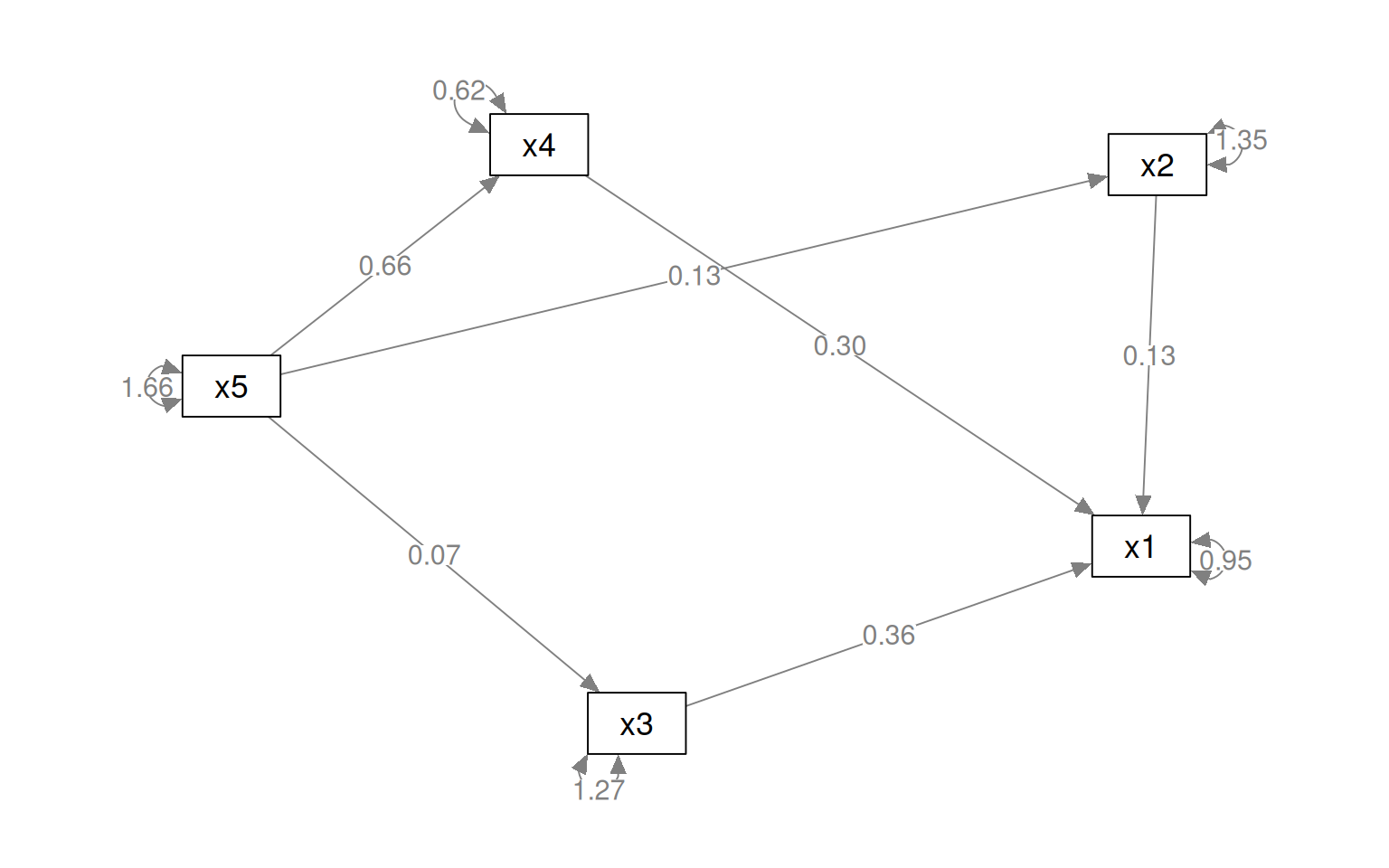Her, Y-M is not significant, but Y-X and M-X are, indicating that M does not mediate the relationship. Note that the model is framed to test whether a particular value is significant–we don’t make two models and compare theme via ANOVA.

## Path analysis and more advanced structural equation modeling

We can use the ~ operator for characterizing a covariance structure among variables, and compare models. The ~ is directional, so we can make complex chains and directional networks. These are really sort of sets of linear regressions. As an example, suppose we want to characterize x1 and being related to 2/3/4, 2 to 4, 3 to 4, but then x1/2/4 all being related to 5:

pathAn1 <- "x1 ~ x2 + x3 + x4
x2 ~ x4
x3 ~ x4
x1 + x2 +x4 ~ x5"

paModel <- sem(pathAn1, data = HolzingerSwineford1939)
summary(paModel, fit.measures = T)
lavaan 0.6-8 ended normally after 16 iterations

Estimator                                         ML
Optimization method                           NLMINB
Number of model parameters                        12

Number of observations                           301

Model Test User Model:

Test statistic                                34.981
Degrees of freedom                                 2
P-value (Chi-square)                           0.000

Model Test Baseline Model:

Test statistic                               392.278
Degrees of freedom                                10
P-value                                        0.000

User Model versus Baseline Model:

Comparative Fit Index (CFI)                    0.914
Tucker-Lewis Index (TLI)                       0.569

Loglikelihood and Information Criteria:

Loglikelihood user model (H0)              -1706.304
Loglikelihood unrestricted model (H1)      -1688.814

Akaike (AIC)                                3436.609
Bayesian (BIC)                              3481.094

Root Mean Square Error of Approximation:

RMSEA                                          0.234
90 Percent confidence interval - lower         0.170
90 Percent confidence interval - upper         0.305
P-value RMSEA <= 0.05                          0.000

Standardized Root Mean Square Residual:

SRMR                                           0.088

Parameter Estimates:

Standard errors                             Standard
Information                                 Expected
Information saturated (h1) model          Structured

Regressions:
Estimate  Std.Err  z-value  P(>|z|)
x1 ~
x2                0.129    0.048    2.685    0.007
x3                0.363    0.050    7.232    0.000
x4                0.249    0.071    3.492    0.000
x2 ~
x4                0.111    0.085    1.311    0.190
x3 ~
x4                0.154    0.055    2.788    0.005
x1 ~
x5                0.059    0.064    0.930    0.353
x2 ~
x5                0.054    0.076    0.704    0.481
x4 ~
x5                0.661    0.035   18.705    0.000

Variances:
Estimate  Std.Err  z-value  P(>|z|)
.x1                0.943    0.077   12.268    0.000
.x2                1.347    0.110   12.268    0.000
.x3                1.243    0.101   12.268    0.000
.x4                0.625    0.051   12.268    0.000
semPaths(paModel, "par", weighted = FALSE, nCharNodes = 7, layout = "spring", sizeMan = 8,
sizeMan2 = 5)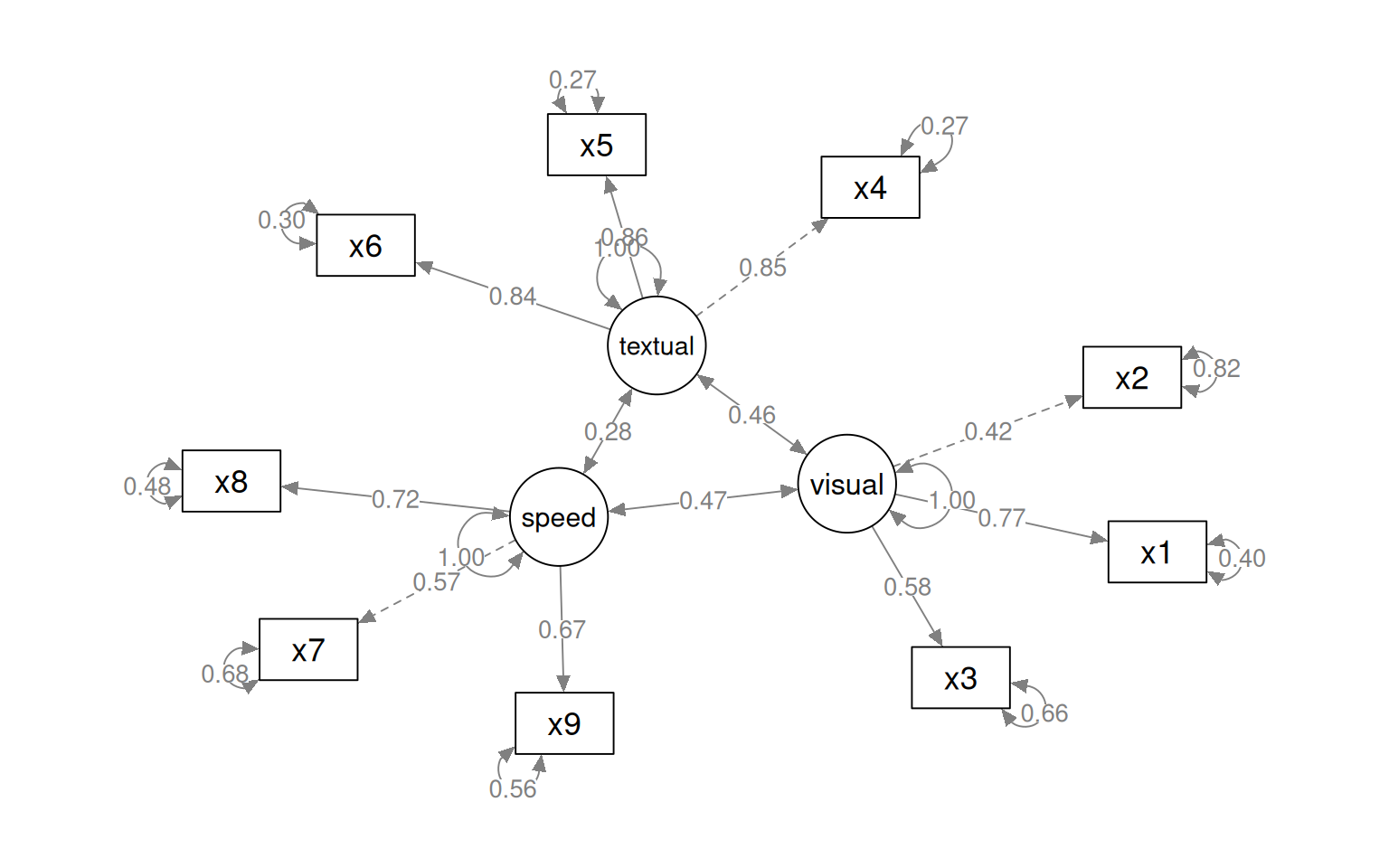This model is not very good (but that is because we are just using it for example data). However, maybe we can try an alternative. This suggests that x1 depends on 2/3/4, and 2/3/4 depend on 5.

pathAn2 <- "x1 ~ x2 + x3 + x4
x2 + x3 + x4 ~ x5"

paModel2 <- sem(pathAn2, data = HolzingerSwineford1939)
summary(paModel2, fit.measures = T)
lavaan 0.6-8 ended normally after 16 iterations

Estimator                                         ML
Optimization method                           NLMINB
Number of model parameters                        10

Number of observations                           301

Model Test User Model:

Test statistic                                43.425
Degrees of freedom                                 4
P-value (Chi-square)                           0.000

Model Test Baseline Model:

Test statistic                               392.278
Degrees of freedom                                10
P-value                                        0.000

User Model versus Baseline Model:

Comparative Fit Index (CFI)                    0.897
Tucker-Lewis Index (TLI)                       0.742

Loglikelihood and Information Criteria:

Loglikelihood user model (H0)              -1710.526
Loglikelihood unrestricted model (H1)      -1688.814

Akaike (AIC)                                3441.053
Bayesian (BIC)                              3478.124

Root Mean Square Error of Approximation:

RMSEA                                          0.181
90 Percent confidence interval - lower         0.135
90 Percent confidence interval - upper         0.231
P-value RMSEA <= 0.05                          0.000

Standardized Root Mean Square Residual:

SRMR                                           0.100

Parameter Estimates:

Standard errors                             Standard
Information                                 Expected
Information saturated (h1) model          Structured

Regressions:
Estimate  Std.Err  z-value  P(>|z|)
x1 ~
x2                0.132    0.048    2.764    0.006
x3                0.359    0.050    7.227    0.000
x4                0.298    0.049    6.135    0.000
x2 ~
x5                0.127    0.052    2.442    0.015
x3 ~
x5                0.068    0.050    1.343    0.179
x4 ~
x5                0.661    0.035   18.705    0.000

Variances:
Estimate  Std.Err  z-value  P(>|z|)
.x1                0.946    0.077   12.268    0.000
.x2                1.355    0.110   12.268    0.000
.x3                1.267    0.103   12.268    0.000
.x4                0.625    0.051   12.268    0.000
semPaths(paModel2, "par", weighted = FALSE, nCharNodes = 7, layout = "spring", sizeMan = 8,
sizeMan2 = 5)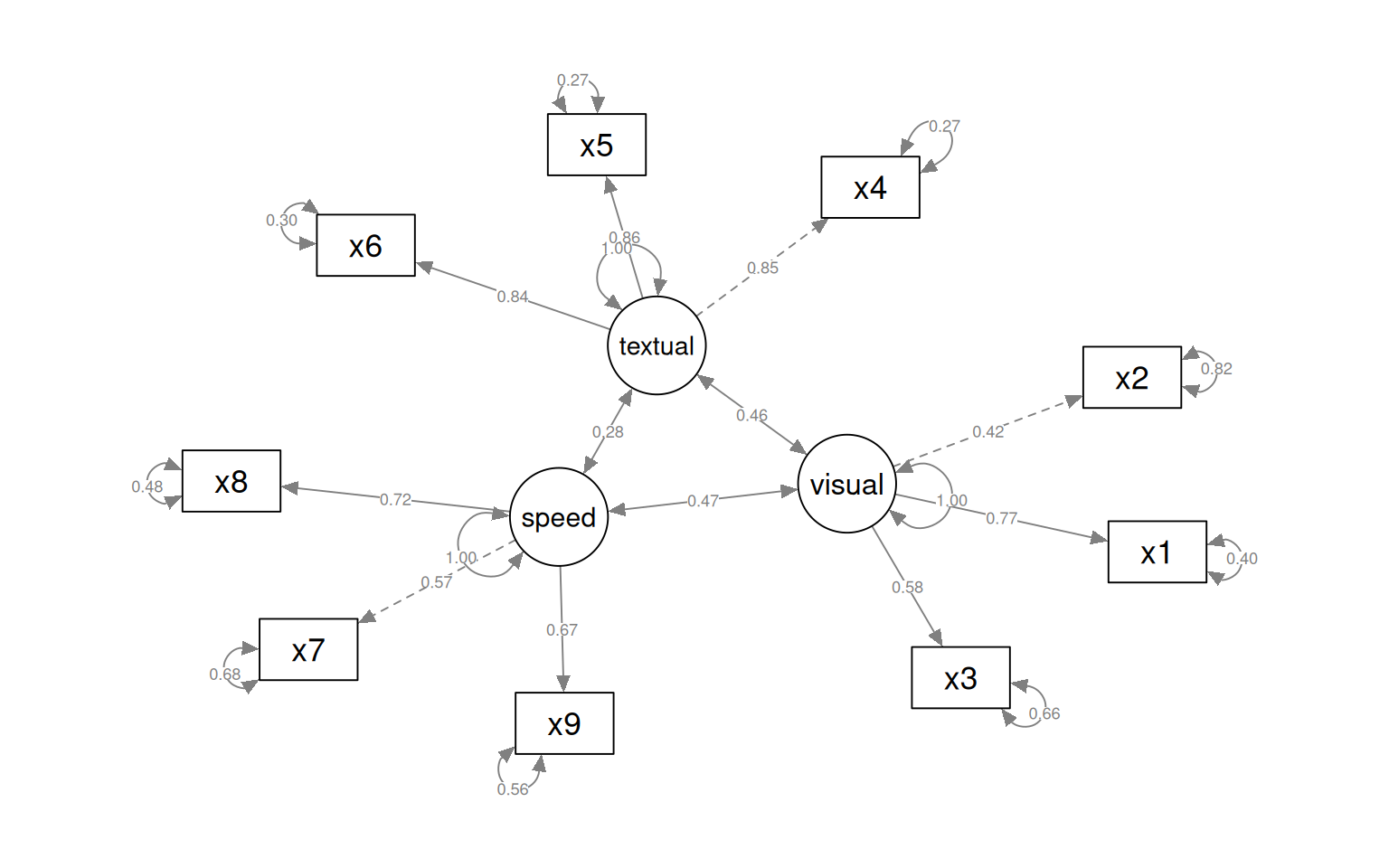anova(paModel, paModel2)
Chi-Squared Difference Test

Df    AIC    BIC  Chisq Chisq diff Df diff Pr(>Chisq)
paModel   2 3436.6 3481.1 34.981
paModel2  4 3441.1 3478.1 43.425     8.4443       2    0.01467 *
---
Signif. codes:  0 '***' 0.001 '**' 0.01 '*' 0.05 '.' 0.1 ' ' 1

There is a significant difference between these, with model2 fitting significantly worse (but being simpler), so we’d prefer to more complex model. Note that that more complex model is not that great.

This is sort of like a simple factor analysis, and let’s you try to find a structural model that describes the relationship between variables, and compare it to other models. However, these models have no latent nodes–they just try to account for the covariance.

We can combine these path-style models with the latent variable models as well. This is a method generally referred to as ‘structural equation modeling’ or SEM.

# Structural Equation Modeling

Structural Equation Modeling (SEM) attempts to divide the model into two parts: the measurement model and an underlying causal model relating the latent variables. The measurement model is sort of like a factor analysis: we have measured variables we want to organize into a single latent construct. The causal model is like path analysis–it models relationships between these latent variables. We will start with the CFA model earlier, but this time fit it with sem:

HS.sem <- " visual   =~ x2 + x1 + x3
textual  =~ x4 + x5 + x6
speed    =~ x7 + x8 + x9 "

fit_sem <- sem(HS.sem, data = HolzingerSwineford1939)
summary(fit_sem, fit.measures = T, standardized = T)
lavaan 0.6-8 ended normally after 38 iterations

Estimator                                         ML
Optimization method                           NLMINB
Number of model parameters                        21

Number of observations                           301

Model Test User Model:

Test statistic                                85.306
Degrees of freedom                                24
P-value (Chi-square)                           0.000

Model Test Baseline Model:

Test statistic                               918.852
Degrees of freedom                                36
P-value                                        0.000

User Model versus Baseline Model:

Comparative Fit Index (CFI)                    0.931
Tucker-Lewis Index (TLI)                       0.896

Loglikelihood and Information Criteria:

Loglikelihood user model (H0)              -3737.745
Loglikelihood unrestricted model (H1)      -3695.092

Akaike (AIC)                                7517.490
Bayesian (BIC)                              7595.339

Root Mean Square Error of Approximation:

RMSEA                                          0.092
90 Percent confidence interval - lower         0.071
90 Percent confidence interval - upper         0.114
P-value RMSEA <= 0.05                          0.001

Standardized Root Mean Square Residual:

SRMR                                           0.065

Parameter Estimates:

Standard errors                             Standard
Information                                 Expected
Information saturated (h1) model          Structured

Latent Variables:
Estimate  Std.Err  z-value  P(>|z|)   Std.lv  Std.all
visual =~
x2                1.000                               0.498    0.424
x1                1.807    0.325    5.554    0.000    0.900    0.772
x3                1.318    0.239    5.509    0.000    0.656    0.581
textual =~
x4                1.000                               0.990    0.852
x5                1.113    0.065   17.014    0.000    1.102    0.855
x6                0.926    0.055   16.703    0.000    0.917    0.838
speed =~
x7                1.000                               0.619    0.570
[ reached getOption("max.print") -- omitted 2 rows ]

Covariances:
Estimate  Std.Err  z-value  P(>|z|)   Std.lv  Std.all
visual ~~
textual           0.226    0.052    4.329    0.000    0.459    0.459
speed             0.145    0.038    3.864    0.000    0.471    0.471
textual ~~
speed             0.173    0.049    3.518    0.000    0.283    0.283

Variances:
Estimate  Std.Err  z-value  P(>|z|)   Std.lv  Std.all
.x2                1.134    0.102   11.146    0.000    1.134    0.821
.x1                0.549    0.114    4.833    0.000    0.549    0.404
.x3                0.844    0.091    9.317    0.000    0.844    0.662
.x4                0.371    0.048    7.778    0.000    0.371    0.275
.x5                0.446    0.058    7.642    0.000    0.446    0.269
.x6                0.356    0.043    8.277    0.000    0.356    0.298
.x7                0.799    0.081    9.823    0.000    0.799    0.676
.x8                0.488    0.074    6.573    0.000    0.488    0.477
.x9                0.566    0.071    8.003    0.000    0.566    0.558
visual            0.248    0.077    3.214    0.001    1.000    1.000
[ reached getOption("max.print") -- omitted 2 rows ]
semPaths(fit_sem, what = "col", whatLabels = "std", style = "mx", rotation = 1, layout = "spring",
nCharNodes = 7, shapeMan = "rectangle", sizeMan = 8, sizeMan2 = 5)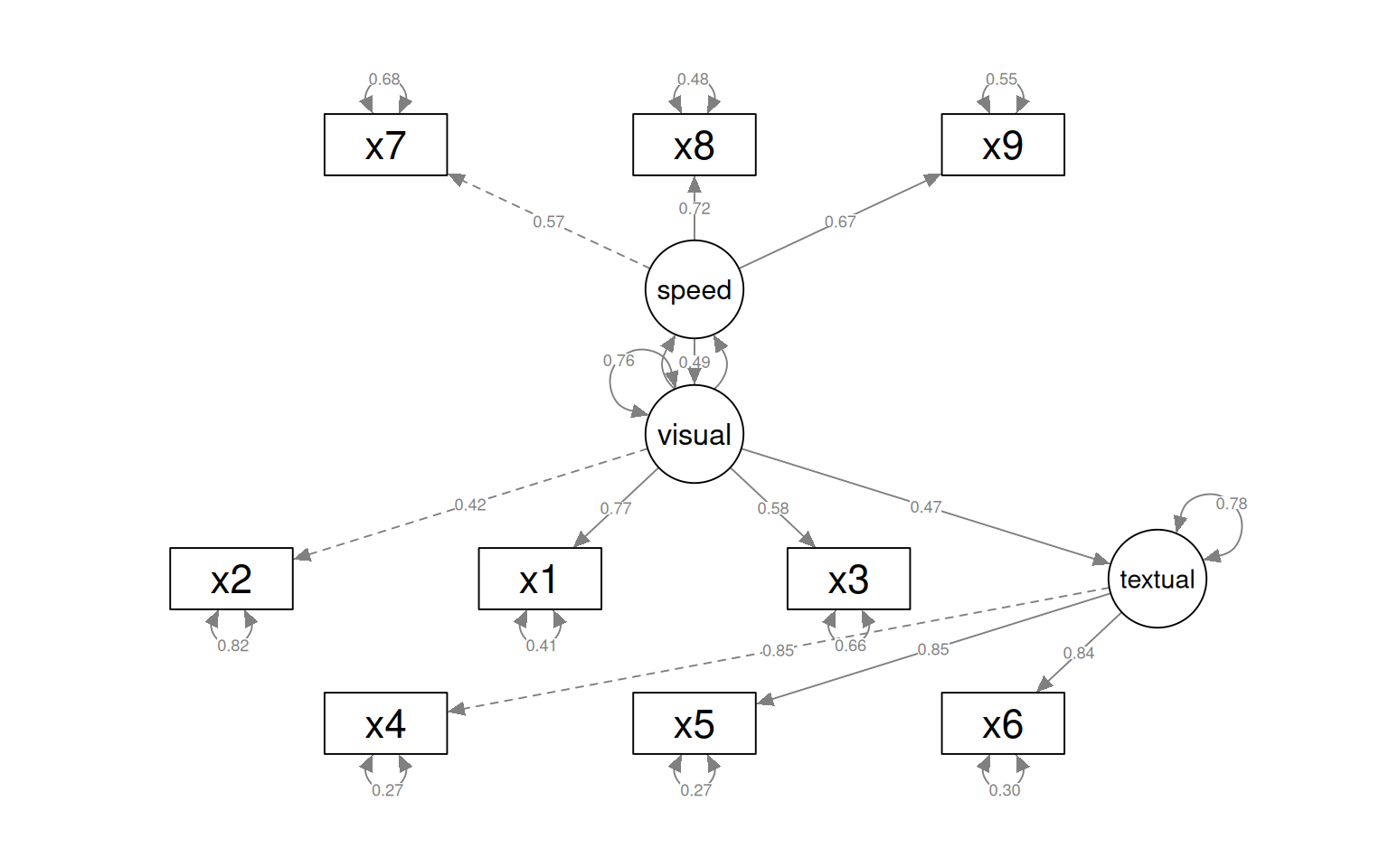With the sem fit, we permit the different factors to have correlations created as bottom-up estimates. This ends up being identical to the cfa solution.

semPaths(fit, what = "col", whatLabels = "std", style = "mx", rotation = 1, layout = "spring",
nCharNodes = 7, shapeMan = "rectangle", sizeMan = 8, sizeMan2 = 5)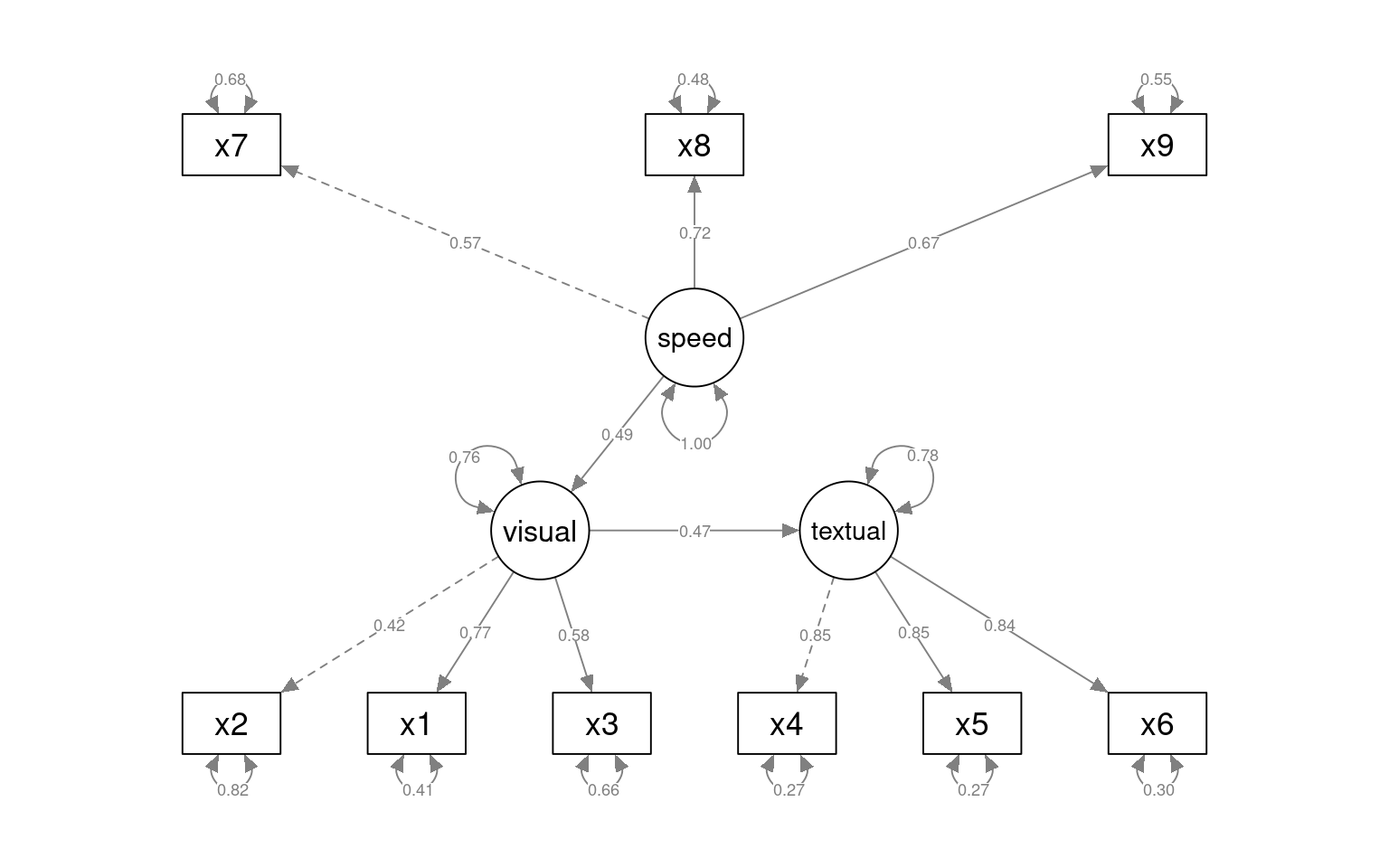We can also specifiy a structural model with ~ notation. Suppose we think that textual is just visual + speed. I’ll force speed and visual to have a fixed variance and allow these to predict textual.

HS.sem2 <- " visual   =~ x2 + x1 + x3
textual  =~ x4 + x5 + x6
speed    =~ x7 + x8 + x9

textual ~ visual + speed
visual ~ 1*visual
speed ~ 1*speed
"

fit_sem2 <- sem(HS.sem2, data = HolzingerSwineford1939)
summary(fit_sem2, fit.measures = T, standardized = T)
lavaan 0.6-8 ended normally after 35 iterations

Estimator                                         ML
Optimization method                           NLMINB
Number of model parameters                        20

Number of observations                           301

Model Test User Model:

Test statistic                               114.128
Degrees of freedom                                25
P-value (Chi-square)                           0.000

Model Test Baseline Model:

Test statistic                               918.852
Degrees of freedom                                36
P-value                                        0.000

User Model versus Baseline Model:

Comparative Fit Index (CFI)                    0.899
Tucker-Lewis Index (TLI)                       0.855

Loglikelihood and Information Criteria:

Loglikelihood user model (H0)              -3752.156
Loglikelihood unrestricted model (H1)      -3695.092

Akaike (AIC)                                7544.313
Bayesian (BIC)                              7618.455

Root Mean Square Error of Approximation:

RMSEA                                          0.109
90 Percent confidence interval - lower         0.089
90 Percent confidence interval - upper         0.129
P-value RMSEA <= 0.05                          0.000

Standardized Root Mean Square Residual:

SRMR                                           0.110

Parameter Estimates:

Standard errors                             Standard
Information                                 Expected
Information saturated (h1) model          Structured

Latent Variables:
Estimate  Std.Err  z-value  P(>|z|)   Std.lv  Std.all
visual =~
x2                1.000                               0.516    0.439
x1                1.732    0.328    5.276    0.000    0.893    0.767
x3                1.260    0.229    5.497    0.000    0.650    0.576
textual =~
x4                1.000                               0.978    0.849
x5                1.113    0.067   16.727    0.000    1.088    0.852
x6                0.926    0.056   16.438    0.000    0.906    0.835
speed =~
x7                1.000                               0.675    0.621
[ reached getOption("max.print") -- omitted 2 rows ]

Regressions:
Estimate  Std.Err  z-value  P(>|z|)   Std.lv  Std.all
textual ~
visual            0.802    0.177    4.527    0.000    0.423    0.423
speed             0.204    0.100    2.046    0.041    0.141    0.141
visual ~
visual            1.000                               1.000    1.000
speed ~
speed             1.000                               1.000    1.000

Variances:
Estimate  Std.Err  z-value  P(>|z|)   Std.lv  Std.all
.x2                1.116    0.103   10.842    0.000    1.116    0.807
.x1                0.560    0.131    4.289    0.000    0.560    0.412
.x3                0.852    0.097    8.804    0.000    0.852    0.668
.x4                0.371    0.048    7.757    0.000    0.371    0.280
.x5                0.447    0.059    7.630    0.000    0.447    0.274
.x6                0.356    0.043    8.252    0.000    0.356    0.302
.x7                0.727    0.085    8.510    0.000    0.727    0.615
.x8                0.398    0.090    4.428    0.000    0.398    0.389
.x9                0.686    0.072    9.595    0.000    0.686    0.676
.visual            0.266    0.082    3.243    0.001    1.000    1.000
[ reached getOption("max.print") -- omitted 2 rows ]
semPaths(fit_sem2, what = "col", whatLabels = "std", style = "mx", rotation = 1,
layout = "tree2", nCharNodes = 7, shapeMan = "rectangle", sizeMan = 8, sizeMan2 = 5)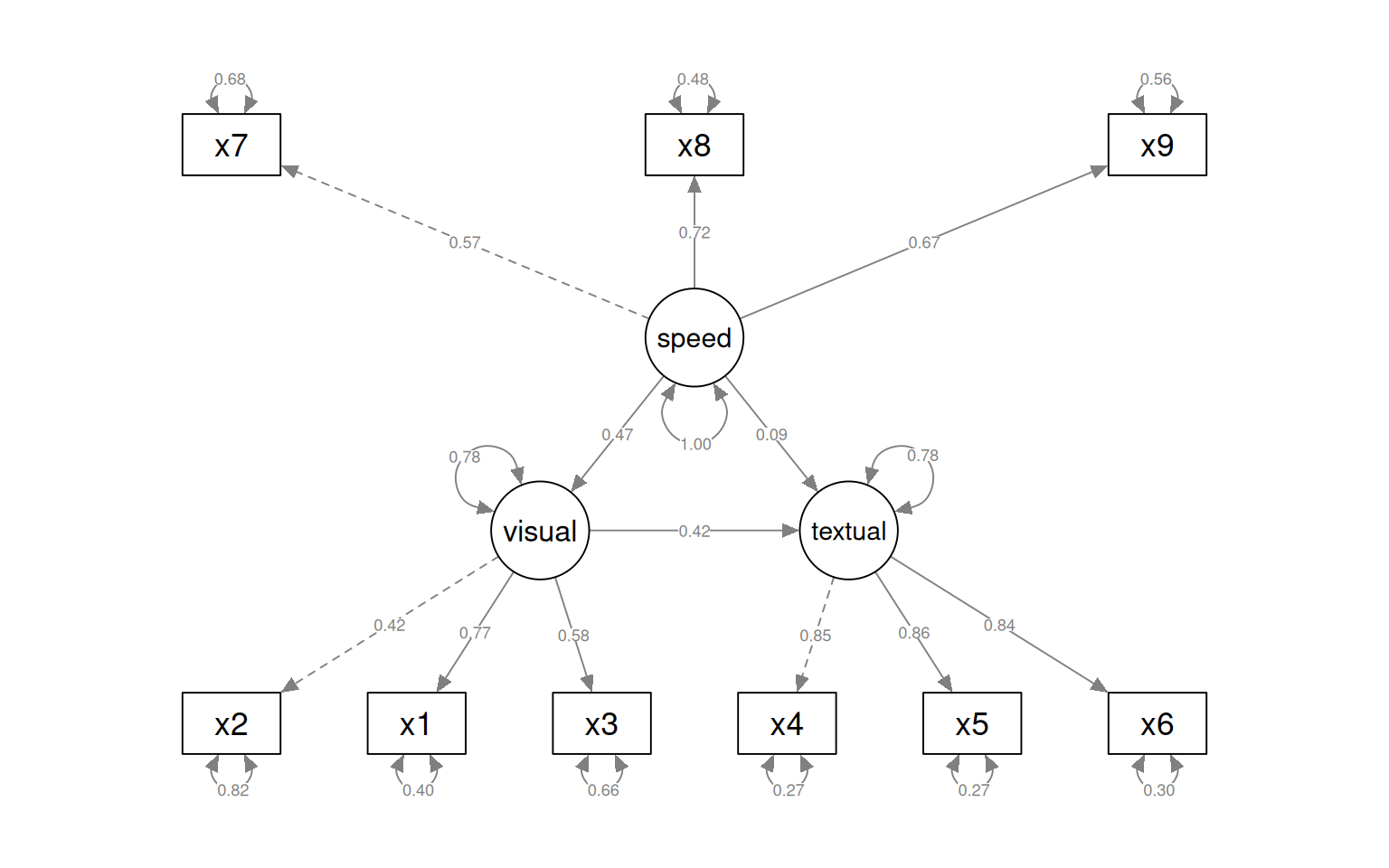We can compare the unconstrained model to the specific causal structure we have specified. Here, we see that the unconstrained model differs significantly via the anova, so we would prefer the more complex model.

anova(fit_sem, fit_sem2)
Chi-Squared Difference Test

Df    AIC    BIC   Chisq Chisq diff Df diff Pr(>Chisq)
fit_sem  24 7517.5 7595.3  85.305
fit_sem2 25 7544.3 7618.5 114.128     28.823       1  7.931e-08 ***
---
Signif. codes:  0 '***' 0.001 '**' 0.01 '*' 0.05 '.' 0.1 ' ' 1